# XBRL Formula Overview 1.0

## Public Working Draft 21 December 2011

This version:
<http://www.xbrl.org/WGN/XBRL-formula-overview/PWD-2011-12-21/XBRL-formula-overview-WGN-PWD-2011-12-21.html>
Editor:
Herm Fischer, Mark V Systems <fischer@markv.com>
Contributors:
Victor Morilla, Banco de España <vmorilla@bde.es>
Bartosz Ochocki, BRAG <bartosz.ochocki@br-ag.eu>
Geoff Shuetrim, Galexy Pty. <geoff@galexy.com>
Masatomo Goto, Fujitsu Ltd. <mg@jp.fujitsu.com>
Roland Hommes, RHOCON <roland@rhocon.nl>
Hugh Wallis, Standard Dimensions <hugh@standarddimensions.com>

## Status

Circulation of this Public Working Draft is unrestricted. Other documents may supersede this document. Recipients are invited to submit comments to formula-feedback@xbrl.org, and to submit notification of any relevant patent rights of which they are aware and provide supporting documentation.

## Abstract

A XBRL formula specifies validations based on XBRL instance facts and generation of derived data as output XBRL instance facts.

This overview provides examples and explanation as an introduction to the syntax and semantics of XBRL formula. The accompanying specifications provide the feature descriptions in a rigorous manner for implementation and validation. Illustrations are based on open source and community edition tools.

### Comment

1 :Should cover these items:

1. - Assertions have a @test, some filters and gen variables have an @select, what is actually ending up in memory when xpath statements are inserted in there. Eg. select count(//xbrli:periodStart) or select @decimals on a generalFilter.
2. - How is the @test xpath being evaluated on the result of the @select? Does whatever is in memory still act as a kind of instance?
3. - What is the syntax for the locationFilter @location?
4. - How come that a parentFilter to xbrli:xbrl gives all facts both on the root AND the facts nested inside a (root based) tuple, but an ancestorFilter on the same instance gives me also the tuples themselves with values of the childconcepts in the tuple?
5. - How do you address xfi:duplicate-tuple? There have to be two variables in there, but how you are making sure that this function iterates over all present tuples and not stumble on comparing the same tuple in both variables?
6. - Same for xfi:duplicate-item
7. - What is the appropriate way to iterate through results of the @select content and treat that outcome as one result?
8. - The @bindAsSequence, how does the memory map look when that is being set to true or false. It looks so many times to have no effect at all at the evaluation being performed.
9. - Is it correct that the variable:function can only be addressed in the @test of the assertion/formula? And that the function is not arc'd to the assertion/formula?

# 1 Introduction

A XBRL formula specifies validations based on XBRL instance facts and generation of derived data as output XBRL instance facts.

This overview provides examples and explanation as an introduction to the syntax and semantics of XBRL formula. The accompanying specifications provide the feature descriptions in a rigorous manner for implementation and validation. Illustrations are based on open source and community edition tools.

# 2 Goals

The goals of XBRL formula are to provide validation capabilities not available in the Base Specification (XBRL 2.1) validation, dimensional validation, in a manner that is closely attuned to XBRL semantics, intuitive in XBRL terms, suitable for business users, extensible, and maintainable. XBRL formula is designed to accommodate the significant variation between filers, annually-released authority instances and filing rules. Finally it is designed to be formally documenting, both for its own maintenance, and to support audit functions.

A goal of XBRL formula is that output instance documents can represent many kinds of derived data, such as transformations, determination of ratios, and data mining.

Transformations can include transforming from one kind of taxonomy to another (for example from Global Ledger to a Financial Reporting taxonomy), from one version of a the same taxonomy to another (such as where namespaces change and some concepts are mapped), and in mapping between different accounting schedules of the same taxonomy (such as relating cash flow to balance sheet and transactions).

Determining of ratios and data mining can identify derived financial data, such as common ratios. In the case of XBRL filings for SEC and IFRS, individual filers may have financial reports that use different concepts or accounting rules than each other. In this case a goal of XBRL formula is to be able to provide declarative specifications that aid in identifying such concepts, which may be by knowing names of alternative names of concepts or possibly by knowing the relationship of concepts to each other (in XBRL's linkbases).

[: Should cover these items:

1. - Assertions have a @test, some filters and gen variables have an @select, what is actually ending up in memory when xpath statements are inserted in there. Eg. select count(//xbrli:periodStart) or select @decimals on a generalFilter.
2. - How is the @test xpath being evaluated on the result of the @select? Does whatever is in memory still act as a kind of instance?
3. - What is the syntax for the locationFilter @location?
4. - How come that a parentFilter to xbrli:xbrl gives all facts both on the root AND the facts nested inside a (root based) tuple, but an ancestorFilter on the same instance gives me also the tuples themselves with values of the childconcepts in the tuple?
5. - How do you address xfi:duplicate-tuple? There have to be two variables in there, but how you are making sure that this function iterates over all present tuples and not stumble on comparing the same tuple in both variables?
6. - Same for xfi:duplicate-item
7. - What is the appropriate way to iterate through results of the @select content and treat that outcome as one result?
8. - The @bindAsSequence, how does the memory map look when that is being set to true or false. It looks so many times to have no effect at all at the evaluation being performed.
9. - Is it correct that the variable:function can only be addressed in the @test of the assertion/formula? And that the function is not arc'd to the assertion/formula?
]

# 3 Other technologies

There are alternative technologies that each meet some goals of XBRL formula. These fall into three areas: procedural programming, declarative programming, and data warehouse/business intelligence.

Procedural programming includes languages common among XBRL processors today, particularly Java and .net languages (C#, and the Visual Basic family of languages). These have robust XML facilities, and in many cases can be coupled with XBRL processors. XBRL processors are generally required for applications that need validated data or deal with XBRL dimensions and semantics. Such processors are mostly implemented in Java, though they have used C# too. These processors have been used, very successfully, to build the XBRL production systems in use today. Meeting formula's goals in this manner requires a significant technical team, generally results in a large sized application, and implies a significant and difficult-to-staff long term maintenance project. In an environment where personnel turnover is likely (such as government contracting), maintenance is risky and often unsuccessful.

Declarative programming includes one language specific to XML, which is XSLT (and a rules-based generator of XSLT called Schematron). Use of XSLT in the 2.0 version (or a custom-modified Schematron implementation) would be required to have access to XBRL's function registry facilities, although there are production systems successful at an earlier time with XBRL 1.0 interfaced to a Java-implemented XBRL processor. XSLT is fully declarative, based on patterns and templates, and in addition has expressions and procedural function capabilities well matched to XBRL's needs. However without capability a function registry, if not available, building XBRL processing at the syntax level is a dauntingly difficult development task (several such projects were successful in XBRL's early days, but no longer are maintainable). XSLT is a difficult language to truely master, and there are very few people who can use the innate raw expressive power that it takes for XBRL processing in XSLT (in contrast to those who use XSLT to simply format reports of traditional XML data, which is relatively easy). Schematron, being a level more abstract than XSLT, is closer to XBRL formula, and this author has semi-automatically migrated Schematron rules specifications to XBRL formula value assertions, so that dimensionally-aware processing could be accommodated.

A declarative programming language specific to XBRL is Sphinx, a private offering by CoreFiling, described as a language for expressing constraints on and between XBRL facts. It can be used to meet the goals of XBRL formula for single input instance, value and existence assertions, but not to produce output instance or transformed instances like XBRL Formula `formula` and `tuple`. Sphinx is a code-based language, with fact accessing constructs reminiscent of XPath element predicates (they call this a primary axis), and square-bracket predicate syntax to specify (filter) based on what XBRL formula calls aspects, e.g., entity, period, unit, explicit and typed dimensions, in a syntax that, though unique, feels (to this author) somewhere between XPath and Python mind-sets. The primary axis and predicate-like filter expressions yield aspect-aware sets on which relational and set functions can be performed. There are nestable iterators remincent of Python-like generator constructs. Sphinx code could be mapped into some portion of XBRL Formula if fact-set operations were added (perhaps supplementing current XPath 2.0 with new operators that, unlike XPath 2.0 general comparators, were `all` rather than `any` operators, and set-level operators with augmentations, such as unit-awareness).

The goal for XBRL formula processing is a specific set of validations, mapping, or derived data to perform for one XBRL input instance (or a set of related input instances to a single process processing formula linkbase). When there are a large (and possibly vast) number of input instances to analyse together, it is likely that the processing capabilities of business warehousing and business intelligence (BW/BI), are a suitable technology. These are usually based on SQL databases, which themselves are a declarative form of programming. Whereas XBRL formula operates directly on native XBRL instances with the full semantics of XBRL, a SQL type of database that underlies BI/BW will need an Extraction, Transformation, and Loading (ETL) process to conform XBRL instance data into the schema structures it requires. ETL for XBRL instances can be complex, as each instance of a vast number (such as SEC filings) is likely to have quite different schematic and semantic structure than another. XBRL instances in BW after ETL will have normalized-away the semantic uniqueness that its DTS had in the original form. XBRL formula's goal is to meet the original form, leaving to BI the goal of performing operations across the reduced set of post-ETL information.

# 4 Formula Processing Overview

The simplified model of formula processing is that an input XBRL instance is provided to a formula processor. The instance specifies discovery of a DTS and includes within it the contexts, units, and facts provided. The formula processor may identify any and all formula linkbase components within that DTS, or it may be given an externally specified formula linkbase. The formula linkbase contains assertions and formulas along with the features to make them work (such as variables, filters and messages). As shown in Figure 1 the processor evaluates some or all assertions and formula processing, and produces assertion results (messages of assertions that are successful or not) and formula results (output XBRL instance facts). In production applications there may be significant interface coding with the formula processor, to control what it should examine, which assertions and formulas may apply to processing inputs, and how to dispose of the formula processing results.

Figure 1: Top level overview of formula processing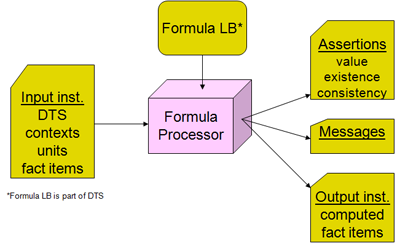There are four formula models, as shown in Figure 2. The first column has the value and existence assertions, which operate on the input XBRL instance data and provide evaluation feedback (as a boolean successful or not successful result, along with possible message detailing cause and ancillary data). The right column has formula which provides a resulting output fact when it is processed, and below is consistency assertion, which is used when it is desired to compare the formula's output fact with a matching one expected in the input XBRL instance.

Figure 2: Four processing models effects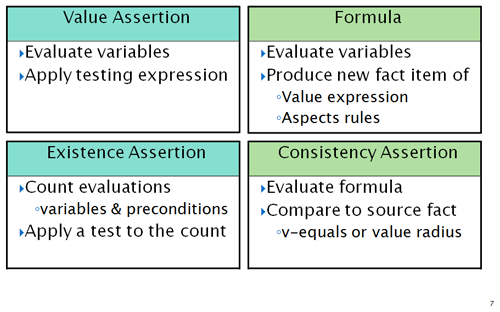Let's first look at simple examples of each of these four models, in Figure 3.

Figure 3: Examples for each processing model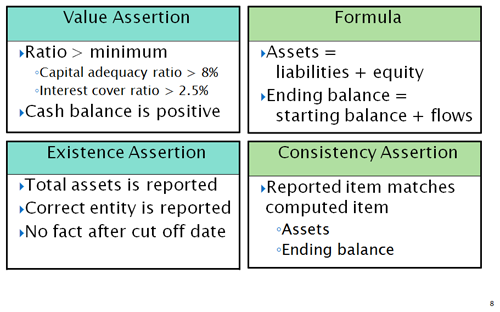Value assertions check expressions, such as might involve arithmetic ratios and algorithms, string and text issues, and date checks. They are coded in XPath 2, and access data of an instance document by declarative filters. They represent the expression being checked, without need to provide plumbing or wiring to associate elements of data to the expression. That is done by the declarative filters which we'll learn of soon.

Existence assertions count the existence of data that the declarative filter expressions were able to locate (or not find) in an input XBRL instance. Existence assertions were meant to simplify expression of data that must be present, but there are situations where the data that is required is complex to specify, dependent on other data present, and requires a message detailing why something was missing due to other present data. In that case a value assertion, which can have an expression based on data evaluated by the filters, may be more suitable than existence, which only counts data evaluations.

Formula is the most sophisticated feature of XBRL formula linkbase. (As one might infer from its name, it was the original first feature of the formula linkbase, before assertions evolved.) Formula is a little like a value assertion, in that it can have an expression, but whereas a value expression is boolean (success or not), a formula expression produces an output XBRL instance fact. The output fact has all of the aspects that are needed to produce output instance XML syntax, including its concept, value (from the fact's expression), precision if numeric, and context/unit aspects. The context and unit aspects can be copied from data that the filters evaluated or constructed by rules. Two situations are common:

1. The output fact is one 'matching' an input fact, or very close to it. Assets = liabilities + equity is such a situation. The formula need only specify the value expression (liabilities + equity), with the filters providing the right data, and ask to copy context and unit information from one of the terms (such as liabilities). The output fact's concept name, assets, would be specified as a rule that overrides that otherwise copied from liabilities. That way the result has the dates, unit, and dimensions of the input term, a different concept name, with barely any programming effort.
2. The output fact is one quite different from input facts, perhaps if a ratio is derived, it may have different units (monetary units per share, something per time), a different period (an ending balance instant period derived from changes that are durations), or quite different dimensions than the source data from which it was derived. That is accommodated by declaratively coded formula rules, instead of programming procedural coding.

A forth processing model is the consistency assertion, which compares a derived formula output fact with the expectation that there is a matching input that should be close enough (within a tolerance margin). Consistency assertion requires a formula, such as producing assets in the figure box above, and a specification of how close in value it should be to the input fact matching it (by percentage or absolute value).

# 5 What's in a formula linkbase

To accomplish formula processing the formula linkbase has a set of components, shown in the expanded view of Figure 4. We'll first identify what the key features are and then begin by example to review them.

Figure 4: Linkbase contents used in XBRL formula processing.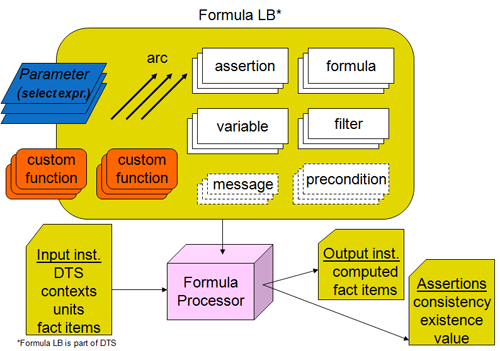Assertions are boolean expressions that are either successful or not, for value (boolean expression evaluation against a matched and possibly filtered set of input fact data), for existence (specified input data exist according to filter expressions), or consistency (a derived output fact is matched to an input fact within a tolerance margin). Formula are values and rule to specify an output fact and its aspects.

Arcs form the relationships that relate the components of a formula linkbase. These relationships are the core of what facilitates extensibility and modularity. Linkbase components that can be shared can have relationships to the sharing features (such as variables and filters shared between different assertions and formulas). These relationships are extensible with the provisions for relationship prohibition. They encourage modularity becase components can be grouped into files for purposes of maintainability and source code management.

Variables declare a way of binding input data, usually fact items, to a name that can be referenced by variable name such as from within an assertion or formula expression. Variables that bind to input fact items are fact variables and use filters to declare what they can bind to in the input. General variables are used for intermediate expression results and other kinds of processing, such as function registry use, lookup table use, and more complex types of DTS linkbase tree traversal.

A variable set is a value assertion, existence assertion, or formula, with its associated variables. The variable set is an evaluatable processing declaration, that when applied by filters to a set of facts in the input XBRL instance, results in assertion attempts and processing of formula values and rules to produce output facts.

Filters specify aspects that constrain which facts can bind to a variable, such as by a name, dimension, or period.

Messages provide text and structured parameters to interface assertion and formula results, with parameters related from evaluation variables, to a reporting or logging system.

Preconditions provide a way of determining if a set of bound variables can activate a formula value and output fact or an assertion value test or existence count.

Parameters are shown overlapping the left border because they can interact with external the external environment in which formula operates. A parameter may have an expression, which is evaluated internally, to produce a global constant for the execution the assertions, formulae, and messages by the processor. It may also have an externally set value. Examples of externally set values would be, for U.S. SEC filings, the expected form type, company identifier, and company name, to be validated against the contents of facts and context information in the input XBRL instance.

Custom functions are shown overlapping the left border because they can be supplied in a programming language implementation that interacts with the external environment. Examples would be custom functions that interact with SQL databases or BI/BW systems. Custom functions can also be implemented within the linkbase (hence the symbols are repeated enclosed in the linkbase). The in-linkbase functions are portable coded in XPath 2 steps, using function arguments, formula parameters, and function registry features, to provide better structure and common expression organization to XBRL formula linkbases.

# 6 Class Models

The figures below provide a model of XBRL formula classes, showing the classes pertinent to variable sets, assertions, formulas producing output facts, and filters, in Figure 5, classes of formula rules in Figure 6, and classes of the filters in Figure 7 and Figure 8.

Figure 5: Variable sets, assertions, formulas, and variables.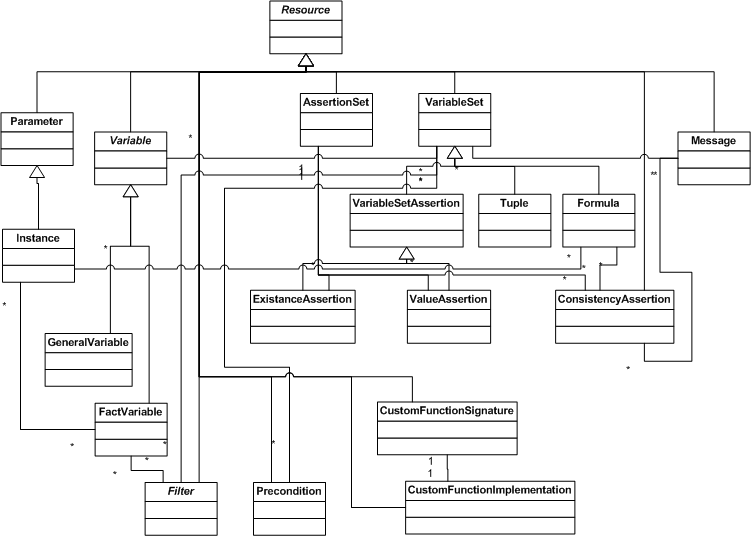Figure 6: Formula Rules Classes.Figure 7: Filter Classes, 1 of 2.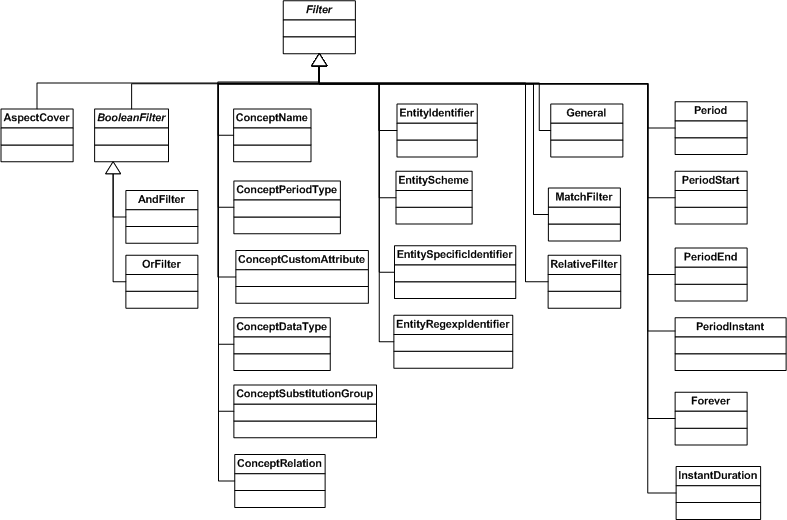Figure 8: Filter Classes, 2 of 2.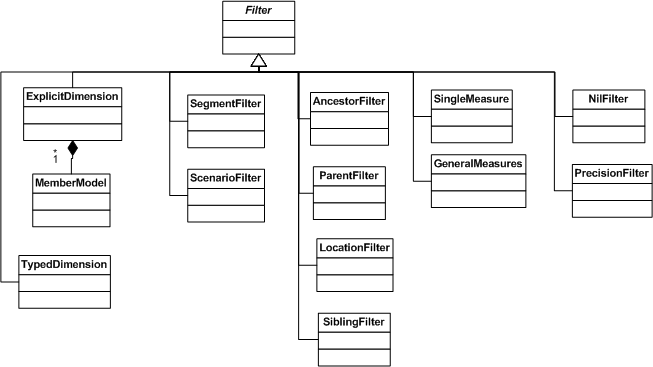# 7 Value assertions

Value assertions often are the most used formula linkbase feature, providing a way to check input XBRL instance facts against an expression. The model in processing value assertions is Figure 9. A formula processor obtains values for any parameters (usually before anything else is done, as they are static during processing). Then it would normally process all the value assertions accessible to the DTS, unless the selection of assertions to process is under the control of an external application. For each value assertion, the variables representing the terms to be evaluated are bound to input XBRL instance facts, according to filtering in effect, and the assertion tested for each applicable set of variables. In this model of processing there are many opportunities for optimisation, such as identifying filter expressions which are common, static, or repeated, and can be removed from repetitive execution, and variable binding in nested iteration conditions, where the nested iterations would duplicate processing from a previous cycle. The illustration, and processing traces below, are simplified to show a serial linear execution (such as is needed for debugging).

Figure 9: Value assertion processing model.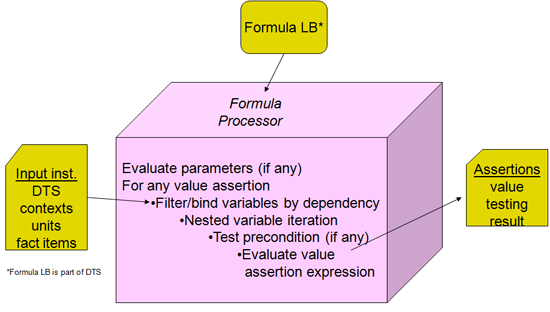## 7.1 Example testing net incomes to gross incomes

The first example tests that net incomes are less than or equal to gross incomes. Both net and gross incomes are reported for two years, facts are shown in Figure 10. For 2007, GrossIncomes is \$500 and NetIncomes is \$200, so the NetIncomes ≤ GrossIncomes is \$200 ≤ \$500 which is true, the assertion should be successful. For 2006, \$1,400 is not ≤ \$900, the assertion should not be successful.

Figure 10: Example net income and gross income facts.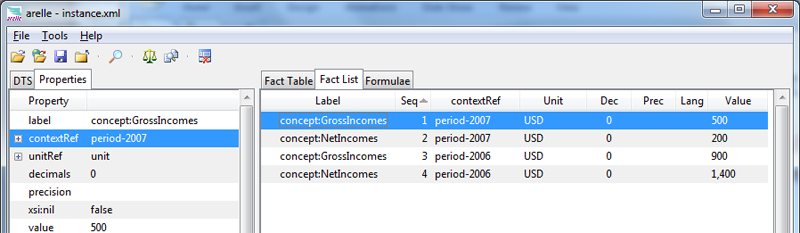A tool-view of the corresponding assertion is shown in Figure 11. The top line is the assertion, expressed with a term for NetIncomes, obtained as a fact from the input XBRL instance, represented in XPath 2 with a dollar symbol before the variable name, \$netIncome. A second term is the corresponding fact item for GrossIncomes, represented by the variable name, \$grossIncomes. The XPath 2 "value comparison" operator for ≤ is the word "le". Each of these terms is, in XBRL formula, a fact variable. Each of these two fact variables declares the concept that they wish to be bound to by a concept name filter, given the QName of the concept, such as concept:NetIncomes. The model for how the assertion declares this is shown in Figure 12

Figure 11: Assertion to test that net incomes are less than gross incomes.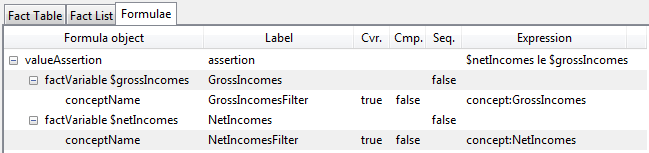A model-view showing how the assertion declares the value assertion expression, its fact variables, and their filters, is shown is shown in Figure 12.

Figure 12: Assertion model showing variables and filters.The value assertion's test expression is `\$netIncomes le \$grossIncomes`. Each of \$netIncomes and \$grossIncomes are specified by fact variables. The fact variables filter (restrict) which variables they may be bound to by concept name filters, each of which specifies a QName that must match the corresponding fact's element name.

In the processing model of Figure 9, the formula processor first identifies assertions to be processed, which is the subject assertion. Next it must bind fact variables to input XBRL instance facts in some order. Relationships are used to identify the fact variables for the assertion. Each such relationship also specifies the variable name to associate with the bound fact. We show the netIncomes being first (but there is no dependency between the two, they could be in any order, even parcelled out to two different CPU cores to run in parallel.

NetIncomes is just a fact variable, if it had no filters, it would iterate through all of the input facts, one by one. However it only makes sense to bind NetIncomes to the two facts for concept:NetIncomes. This is specified by the concept name filter, which has a variable-filter relationship to the fact variable. The use of relationships to connect assertions to fact variables to filters provides the flexibility to share common variables (and filters) between different assertions (with name given by the relationship, so that a multiply-used variable can have names specified on each such relationship.

Once the first fact variable, NetIncomes, is bound to a fact then the second fact variable likewise is bound to a fact, but implicit filtering narrows down the scope of which of the GrossIncomes facts can correspond to each NetIncomes fact. The result of implicit filtering is that the 2007 facts represent one evaluation (assignment of value to each variable and trying out the assertion value expression), and the 2006 facts represent the other evaluation.

With facts bound to both NetIncomes and GrossIncomes, the assertion can be evaluated. After the evaluation the processing model of Figure 9 first attempts to be sure that there aren't more GrossIncomes that can be evaluated. There is one more GrossIncomes fact, but it doesn't match NetIncomes in the period aspect (by implicit filtering), so it's skipped.

Once the other GrossIncomes facts had been considered (but not evaluated because of implicit filtering periods mismatch), then the NetIncomes fact variable can assume the second value, and the GrossIncomes tried again.

Example 1 is a detailed trace of the execution of a formula processor with the above instance and assertion (using the Arelle open source processor).

Example 1: Assertion execution detailed trace

Trace log Meaning
`Fact Variable netIncomes filtering: start with 4 facts` Processing of the assertion begins by finding a first fact to bind to \$netIncomes. All four facts in the instance are, at first, candidates to be bound (one by one) to \$netIncomes.
`Fact Variable netIncomes conceptName filter NetIncomesFilter passes 2 facts` The concept name filter that is associated to this fact variable only passes the two facts which have QName matching `concept:NetIncomes`
`Fact Variable netIncomes: filters result [fact(concept:NetIncomes, period-2007, unit, '200'), fact(concept:NetIncomes, period-2006, unit, '1,400')]` Now these two filter result facts are bound, one by one, to \$netIncomes.
`Fact Variable netIncomes: bound value fact(concept:NetIncomes, period-2007, unit, '200')` First of two netIncomes fact items is bound to \$netIncomes.
`Fact Variable grossIncomes filtering: start with 4 facts` GrossIncome starts with all 4 facts in the instance
`Fact Variable grossIncomes conceptName filter GrossIncomesFilter passes 2 facts` The concept name filter that is associated to this fact variable only passes the two facts which have QName matching `concept:GrossIncomes`
`Fact Variable netIncomes implicit filter period passes 1 facts` Implicit filtering considers all uncovered aspects of the gross income fact, trying to match it to the net income fact. In this example we focus on the period aspect (skipping the traces of aspects that aren't material to this example)
`Fact Variable grossIncomes: filters result [fact(concept:GrossIncomes, period-2007, unit, '500')]` Only one of the gross income facts matched the period of the net income fact, and survives this filter>
`Fact Variable grossIncomes: bound value fact(concept:GrossIncomes, period-2007, unit, '500')`
`Value Assertion assertion ` Now both net income and gross income have bound values, and the assertion expression can be evaluated
`Result: True`
Given the evaluation of the assertion, if there were more than one fact assignable to \$grossIncomes it would now be assigned and evaluated, but only one grossIncome fact had been found to pass the filters and implicitly match (by period) to net incomes, so next processing tries for another value of the next outer (first) fact fact variable.
`Fact Variable netIncomes: bound value fact(concept:NetIncomes, period-2006, unit, '1,400')` Second of two netIncomes fact items is bound to \$netIncomes.
`Fact Variable grossIncomes filtering: start with 4 facts`
`Fact Variable grossIncomes conceptName filter GrossIncomesFilter passes 2 facts`
`Fact Variable netIncomes implicit filter period passes 1 facts` Second gross income is period matched to second net income fact
`Fact Variable grossIncomes: filters result [fact(concept:GrossIncomes, period-2006, unit, '900')]`
`Fact Variable grossIncomes: bound value fact(concept:GrossIncomes, period-2006, unit, '900')`
`Value Assertion assertion `
`Result: False` Second assertion result is false as expected from the data
`Value Assertion assertion evaluations : 1 satisfied, 1 not satisfied`

Example 2 shows the syntax of the linkbase.

Example 2: Assertion example linkbase syntax

`<generic:link xlink:type="extended" xlink:role="http://www.xbrl.org/2003/role/link">` An extended link is the container for formula linkbase resources and arcs. The xlink:role is not significant for formula linkbases, and is not used to separate or control assertion execution (see assertion sets for partitioning of assertions into managed sets)
``` <va:valueAssertion xlink:type="resource" xlink:label="assertion" id="assertion" aspectModel="dimensional" implicitFiltering="true" test="\$netIncomes le \$grossIncomes"/> ``` This is the value assertion. Its xlink:label is the source of arcs to the fact variables of the value assertion. The aspect model, dimensional, specifies that dimensions may be used as aspects on facts (and that implicit filtering will match up uncovered dimension aspects, if there were any in this example).
``` <variable:factVariable xlink:type="resource" xlink:label="GrossIncomes" bindAsSequence="false"/> ``` Specifies the fact variable for gross incomes, but does not assign the 'variable name' (that is done on the arc from the assertion to the fact variable). The filtered facts that are assigned to this fact variable are processed one-by-one because bindAsSequence is false.
``` <variable:factVariable xlink:type="resource" xlink:label="NetIncomes" bindAsSequence="false"/> ``` Ditto, the fact variable for net incomes.
``` <variable:variableArc xlink:type="arc" xlink:arcrole="http://xbrl.org/arcrole/2008/variable-set" xlink:from="assertion" xlink:to="GrossIncomes" order="1.0" name="grossIncomes"/> ``` This arc, from the assertion to the fact variable, gives the fact variable its name when used on this assertion (\$grossIncomes). (If the fact variable were to have been shared with other assertions, it could have been given different names on the arc from those other assertions.)
``` <variable:variableArc xlink:type="arc" xlink:arcrole="http://xbrl.org/arcrole/2008/variable-set" xlink:from="assertion" xlink:to="NetIncomes" order="2.0" name="netIncomes"/> ``` Ditto, arc from assertion to net incomes fact variable, assigning it the name (\$netIncomes).
``` <cf:conceptName xlink:type="resource" xlink:label="GrossIncomesFilter"><cf:concept><cf:qname> concept:GrossIncomes </cf:qname></cf:concept></cf:conceptName> ``` The filter used by the fact variable for gross incomes, to pass facts which have the concept name, concept:GrossIncomes, and to reject any other named facts.
``` <cf:conceptName xlink:type="resource" xlink:label="NetIncomesFilter"><cf:concept><cf:qname> concept:NetIncomes </cf:qname></cf:concept></cf:conceptName> ``` Ditto, for net incomes.
``` <variable:variableFilterArc xlink:type="arc" xlink:arcrole="http://xbrl.org/arcrole/2008/variable-filter" xlink:from="GrossIncomes" xlink:to="GrossIncomesFilter" complement="false" cover="true" order="1.0"/> ``` The arc from fact variable gross incomes to its filter (restricting to concepts named Gross incomes).
``` <variable:variableFilterArc xlink:type="arc" xlink:arcrole="http://xbrl.org/arcrole/2008/variable-filter" xlink:from="NetIncomes" xlink:to="NetIncomesFilter" complement="false" cover="true" order="1.0"/> ``` Ditto for net incomes.
`</generic:link>` End of extended link formula resources and arcs construct.

## 7.2 Example testing movement balances

This example tests a movement pattern, where there is a starting balance, changes, and ending balance, for each of several periods, and it is desired to validate that the ending balance is within a tolerance margin of the starting balance plus changes. The XBRL calculation linkbase doesn't apply to this situation because the balances are instant periods and the changes are duration periods, so a value assertion is required.

Example facts are shown in Figure 13. For 2008, \$600 + \$400 = \$1000, the assertion is successful. For 2009, \$1,000 + \$800 ≠ \$1,790 (within tolerance of \$1), so this assertion is unsuccessful. For 2010, \$1,790 + \$900 = \$2,690 so this assertion is successful.

Figure 13: Example movement balance and changes facts.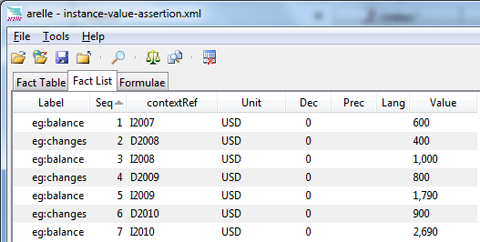The corresponding assertion is shown in Figure 14. The top line is the assertion, \$beginningBalance + \$changes = \$endingBalance within tolerance margin (1.00). This is coded as | \$beginningBalance + \$changes - \$endingBalance | ≤ 1.00, or in XPath 2, abs( \$beginningBalance + \$changes - \$endingBalance ) le 1.00. This example introduces explicit filtering for the period aspect of the balances (compared to the prior example, where period filtering was done implicitly). Explicit filtering is required to establish that the starting balance instant period matches the start of the changes duration period, and the ending balance likewise matches the end of the changes period.

Figure 14: Assertion to test starting balance, changes, and ending balance.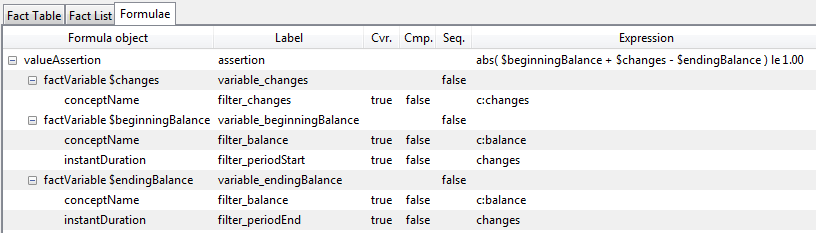A model-view showing how the assertion declares the value assertion expression, its fact variables, and their filters, is shown is shown in Figure 15.

Figure 15: Assertion model showing variables and filters.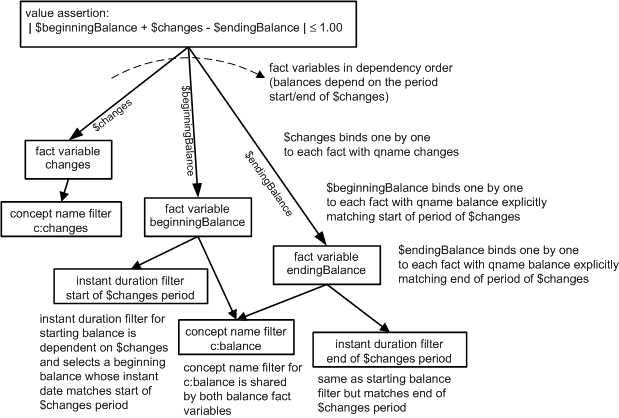This example has a dependency between the changes and balance fact variables, the changes fact must be bound first, so that the balances for start and end can respectively bind to the start and end dates of the period of the changes variable. Also the diagram shows how filters may be shared, as the same concept name filter is used for both balance fact variables.

Example 3 is a detailed trace of the execution of a formula processor with the above instance and assertion (using the Arelle open source processor).

Example 3: Assertion execution detailed trace

Trace log Meaning
`Fact Variable changes filtering: start with 7 facts` \$changes starts with all facts in instance
`Fact Variable changes conceptName filter filter_changes passes 3 facts` concept name filtering by QName c:changes
`Fact Variable changes: filters result [fact(concept:changes, D2008, unit, '400'), fact(concept:changes, D2009, unit, '800'), fact(concept:changes, D2010, unit, '900')]` 3 resulting changes facts (to be bound to \$changes one-by-one)
`Fact Variable changes: bound value fact(concept:changes, D2008, unit, '400')` First evaluation with 2008's changes fact
`Fact Variable beginningBalance filtering: start with 7 facts` \$beginningBalance starts with all facts
`Fact Variable beginningBalance conceptName filter filter_balance passes 4 facts` concept name filtering by c:balance
`Fact Variable beginningBalance instantDuration filter filter_periodStart passes 1 facts` period start filter to match \$changes start filters down to one fact
`Fact Variable beginningBalance: filters result [fact(concept:balance, I2007, unit, '600')]`
`Fact Variable beginningBalance: bound value fact(concept:balance, I2007, unit, '600')`
`Fact Variable endingBalance filtering: start with 7 facts` (same as beginning balance)
`Fact Variable endingBalance conceptName filter filter_balance passes 4 facts`
`Fact Variable endingBalance instantDuration filter filter_periodEnd passes 1 facts` (but matches \$changes end date)
`Fact Variable endingBalance: filters result [fact(concept:balance, I2008, unit, '1,000')]`
`Fact Variable endingBalance: bound value fact(concept:balance, I2008, unit, '1,000')`
```Value Assertion assertion Result: True``` Assertion is successful for 2008 changes.
`Fact Variable changes: bound value fact(concept:changes, D2009, unit, '800')` Processing continues with \$changes binding to the second of its facts, for 2009 (the order of taking changes facts is arbitrary and not important, this processor is using document order which happens to be in year order).
`Fact Variable beginningBalance filtering: start with 7 facts`
`Fact Variable beginningBalance conceptName filter filter_balance passes 4 facts`
`Fact Variable beginningBalance instantDuration filter filter_periodStart passes 1 facts`
`Fact Variable beginningBalance: filters result [fact(concept:balance, I2008, unit, '1,000')]`
`Fact Variable beginningBalance: bound value fact(concept:balance, I2008, unit, '1,000')`
`Fact Variable endingBalance filtering: start with 7 facts`
`Fact Variable endingBalance conceptName filter filter_balance passes 4 facts`
`Fact Variable endingBalance instantDuration filter filter_periodEnd passes 1 facts`
`Fact Variable endingBalance: filters result [fact(concept:balance, I2009, unit, '1,790')]`
`Fact Variable endingBalance: bound value fact(concept:balance, I2009, unit, '1,790')`
```Value Assertion assertion Result: False```
`Fact Variable changes: bound value fact(concept:changes, D2010, unit, '900')`
`Fact Variable beginningBalance filtering: start with 7 facts`
`Fact Variable beginningBalance conceptName filter filter_balance passes 4 facts`
`Fact Variable beginningBalance instantDuration filter filter_periodStart passes 1 facts`
`Fact Variable beginningBalance: filters result [fact(concept:balance, I2009, unit, '1,790')]`
`Fact Variable beginningBalance: bound value fact(concept:balance, I2009, unit, '1,790')`
`Fact Variable endingBalance filtering: start with 7 facts`
`Fact Variable endingBalance conceptName filter filter_balance passes 4 facts`
`Fact Variable endingBalance instantDuration filter filter_periodEnd passes 1 facts`
`Fact Variable endingBalance: filters result [fact(concept:balance, I2010, unit, '2,690')]`
`Fact Variable endingBalance: bound value fact(concept:balance, I2010, unit, '2,690')`
```Value Assertion assertion Result: True```
`Value Assertion assertion evaluations : 2 satisfied, 1 not satisfied`

Example 4 shows the syntax of the linkbase.

Example 4: Assertion example linkbase syntax

``` <va:valueAssertion test="abs( \$beginningBalance + \$changes - \$endingBalance ) le 1.00"/> ``` Value assertion with the balances changes testing XPath expression
``` <variable:factVariable xlink:type="resource" xlink:label="variable_beginningBalance" bindAsSequence="false"/> ``` Beginning balance fact variable
``` <variable:factVariable xlink:type="resource" xlink:label="variable_endingBalance" bindAsSequence="false"/> ``` Ending balance fact variable
``` <variable:factVariable xlink:type="resource" xlink:label="variable_changes" bindAsSequence="false"/> ``` Changes fact variable
``` <variable:variableArc xlink:type="arc" xlink:arcrole="http://xbrl.org/arcrole/2008/variable-set" xlink:from="assertion" xlink:to="variable_changes" order="1.0" name="changes"/> ``` Relationships from assertion to the fact variables
``` <variable:variableArc xlink:type="arc" xlink:arcrole="http://xbrl.org/arcrole/2008/variable-set" xlink:from="assertion" xlink:to="variable_beginningBalance" order="2.0" name="beginningBalance"/> ```
``` <variable:variableArc xlink:type="arc" xlink:arcrole="http://xbrl.org/arcrole/2008/variable-set" xlink:from="assertion" xlink:to="variable_endingBalance" order="2.0" name="endingBalance"/> ```
``` <cf:conceptName xlink:type="resource" xlink:label="filter_balance"><cf:concept><cf:qname>c:balance</cf:qname></cf:concept></cf:conceptName> ``` Shared concept name filter for both balances fact variables
``` <cf:conceptName xlink:type="resource" xlink:label="filter_changes"><cf:concept><cf:qname>c:changes</cf:qname></cf:concept></cf:conceptName> ``` Concept name filter for changes fact variable
``` <variable:variableFilterArc xlink:type="arc" xlink:arcrole="http://xbrl.org/arcrole/2008/variable-filter" complement="false" cover="true" xlink:from="variable_changes" xlink:to="filter_changes" order="1.0"/> ``` Changes fact variable concept name filter relationship
``` <variable:variableFilterArc xlink:type="arc" xlink:arcrole="http://xbrl.org/arcrole/2008/variable-filter" complement="false" cover="true" xlink:from="variable_beginningBalance" xlink:to="filter_balance" order="1.0"/> ``` Beginning balance fact variable shared concept name filter relationship
``` <variable:variableFilterArc xlink:type="arc" xlink:arcrole="http://xbrl.org/arcrole/2008/variable-filter" complement="false" cover="true" xlink:from="variable_endingBalance" xlink:to="filter_balance" order="1.0"/> ``` Ending balance fact variable shared concept name filter relationship
``` <pf:instantDuration xlink:type="resource" xlink:label="filter_periodStart" variable="changes" boundary="start"/> ``` Period start filter aligning beginning balance to start of \$changes duration period
``` <pf:instantDuration xlink:type="resource" xlink:label="filter_periodEnd" variable="changes" boundary="end"/> ``` (same for ending balance)
``` <variable:variableFilterArc xlink:type="arc" xlink:arcrole="http://xbrl.org/arcrole/2008/variable-filter" complement="false" cover="true" xlink:from="variable_beginningBalance" xlink:to="filter_periodStart" order="6.0"/> ``` Beginning balance fact variable period filter relationship
``` <variable:variableFilterArc xlink:type="arc" xlink:arcrole="http://xbrl.org/arcrole/2008/variable-filter" complement="false" cover="true" xlink:from="variable_endingBalance" xlink:to="filter_periodEnd" order="6.0"/> ```

## 7.3 Dimensional examples

This example tests a dimensional example, adapted from Victor Morilla's U-Tube XBRL Formula presentation to XBRL 22 in Brussels. Example facts are shown in Figure 16. For a single year, balance sheet facts are shown for a default total dimension, as well as breakdowns by Europe and USA, and within Europe an incomplete breakdown of just France, Germany, and Spain. We will use this dimensional example to show implicit filtering by dimensional aspects, validation formulas with dimensions, and dimensional aggregation. A fact list view, showing concepts (which are dimensional) is in Figure 17, and shows the style of concept usage for dimensional values. The total dimension is default (not appearing in contexts).

Figure 16: Example dimensional facts table by dimension.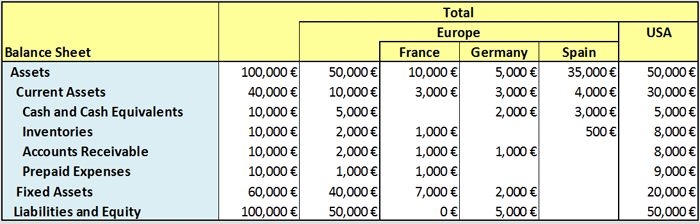Figure 17: Example dimensional fact list by contextRef.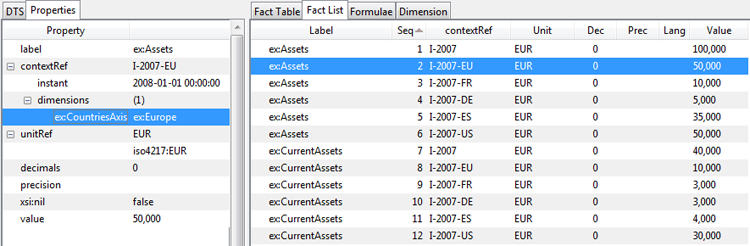### 7.3.1 Testing assets equals liabilities and equity per dimension

This first assertion tests that assets = liabilitiesAndEquities in each dimension. The model of the assertion is in Figure 18. There are two fact variables, the first binding to assets one by one. Each assets fact has a default or explicit member value for the CountriesAxis dimension. The second fact variable is implicitly matched to all uncovered aspects of \$assets, in particular the Country dimension. As seen in Figure 16, for the France entry, the liabilities and equity is 0€, compared to assets of 10,000€, so the France assertion fails. Spain has no entry for liabilitiesAndEquity, causing the evaluation to not bind (see trace below), and thus no assertion is attempted for Spain.

Figure 18: Assertion to test Assets to LiabilitiesAndEquity in each dimension.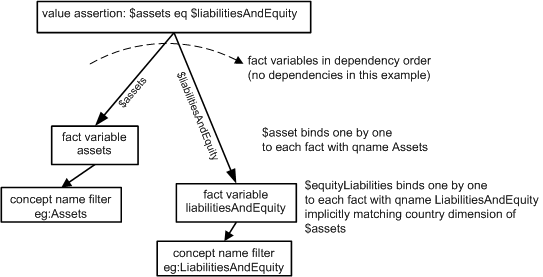Example 3 is an excerpt of the trace of the execution of a formula processor with the above instance and assertion.

Example 5: Assertion execution trace excerpt

Trace log Meaning
`Fact Variable assets filtering: start with 41 facts` Assets starts with all facts in instance
`Fact Variable assets conceptName filter filter_assets passes 6 facts`
`Fact Variable assets: filters result [fact(ex:Assets, I-2007, EUR, '100,000'), fact(ex:Assets, I-2007-EU, EUR, '50,000'), fact(ex:Assets, I-2007-FR, EUR, '10,000'), fact(ex:Assets, I-2007-DE, EUR, '5,000'), fact(ex:Assets, I-2007-ES, EUR, '35,000'), fact(ex:Assets, I-2007-US, EUR, '50,000')]` Now bound only to Assets facts
`Fact Variable assets: bound value fact(ex:Assets, I-2007, EUR, '100,000')` Bound one by one to each Assets fact
`Fact Variable liabilitiesAndEquity filtering: start with 41 facts`
`Fact Variable liabilitiesAndEquity conceptName filter filter_liabilitiesAndEquity passes 5 facts`
`Fact Variable assets implicit filter ex:CountriesAxis passes 1 facts` Implicit match of CountriesAxis dimension matches LiabilitiesAndEquity to dimension of Assets
`Fact Variable liabilitiesAndEquity: filters result [fact(ex:LiabilitiesAndEquity, I-2007, EUR, '100,000')]`
`Fact Variable liabilitiesAndEquity: bound value fact(ex:LiabilitiesAndEquity, I-2007, EUR, '100,000')`
```Value Assertion assertion Result: True```
`Fact Variable assets: bound value fact(ex:Assets, I-2007-EU, EUR, '50,000')` The next country axis dimension member (skipping detailed trace steps)
```Value Assertion assertion Result: True```
`Fact Variable assets: bound value fact(ex:Assets, I-2007-FR, EUR, '10,000')` France Assets
`Fact Variable liabilitiesAndEquity filtering: start with 41 facts`
`Fact Variable liabilitiesAndEquity conceptName filter filter_liabilitiesAndEquity passes 5 facts`
`Fact Variable assets implicit filter ex:CountriesAxis passes 1 facts`
`Fact Variable liabilitiesAndEquity: filters result [fact(ex:LiabilitiesAndEquity, I-2007-FR, EUR, '0')]`
`Fact Variable liabilitiesAndEquity: bound value fact(ex:LiabilitiesAndEquity, I-2007-FR, EUR, '0')` France LiabilitiesAndEquity is zero, doesn't match Assets
```Value Assertion assertion Result: False``` Fails because liabilities value is 0, compared with 10,000 for Assets
`Fact Variable assets: bound value fact(ex:Assets, I-2007-DE, EUR, '5,000')`
`Fact Variable liabilitiesAndEquity filtering: start with 41 facts` Germany, details omitted
```Value Assertion assertion Result: True```
`Fact Variable assets: bound value fact(ex:Assets, I-2007-ES, EUR, '35,000')` Spain has Assets but no LiabilitiesAndEquity fact
`Fact Variable liabilitiesAndEquity filtering: start with 41 facts`
`Fact Variable liabilitiesAndEquity conceptName filter filter_liabilitiesAndEquity passes 5 facts`
`Fact Variable assets implicit filter ex:CountriesAxis passes 0 facts` No country axis Spain member found to match Assets for Spain
`Fact Variable liabilitiesAndEquity: filters result []` Empty sequence results for Spain, note that there is no evaluation of the assertion because the second fact variable failed to bind.
`Fact Variable assets: bound value fact(ex:Assets, I-2007-US, EUR, '50,000')` Now trying USA dimension member
`Fact Variable liabilitiesAndEquity filtering: start with 41 facts`
`Fact Variable assets implicit filter ex:CountriesAxis passes 1 facts`
`Fact Variable liabilitiesAndEquity: filters result [fact(ex:LiabilitiesAndEquity, I-2007-US, EUR, '50,000')]`
`Fact Variable liabilitiesAndEquity: bound value fact(ex:LiabilitiesAndEquity, I-2007-US, EUR, '50,000')`
```Value Assertion assertion Result: True``` Successful for USA dimension member
`Value Assertion assertion evaluations : 4 satisfied, 1 not satisfied`

### 7.3.2 Testing assets equals current assets plus fixed assets with fallback

This assertion introduces fallback values for missing facts while testing that assets = currentAssets + fixedAssets for each dimension. Review of the facts table for Spain in Figure 16 shows that the entry for fixedAssets is missing. Without a fallback for the fact variable, no evaluation would occur (as above for Spain). However here we desire an unsuccessful evaluation, which can be caused by providing a declaration that in the absence of a value for the fact variable, it is to fallback to zero.
Example 6 The model of the assertion is in Figure 19.

Example 6: Fallback value fact variables example linkbase syntax

``` <variable:factVariable xlink:type="resource" xlink:label="variable_assets" bindAsSequence="false" fallbackValue="0"/> ``` Fact variables each specify fallbackValue="0"
``` <variable:factVariable xlink:type="resource" xlink:label="variable_currentAssets" bindAsSequence="false" fallbackValue="0"/> ```
``` <variable:factVariable xlink:type="resource" xlink:label="variable_fixedAssets" bindAsSequence="false" fallbackValue="0"/> ```
Figure 19: Assertion to test Assets to LiabilitiesAndEquity in each dimension.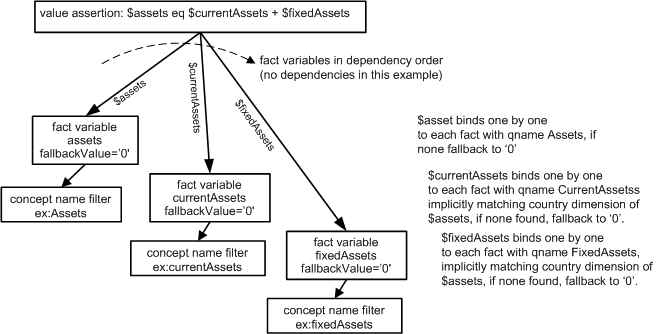Example 7 is an excerpt of the trace of the execution of a formula processor with the above instance and assertion.

Example 7: Assertion execution trace excerpt with fallback value

Trace log Meaning
```Value Assertion assertion Result: True ``` Total (default country dimension member)
```Value Assertion assertion Result: True ``` Europe
```Value Assertion assertion Result: True ``` France
```Value Assertion assertion Result: True ``` Germany
```Value Assertion assertion Result: True ``` USA
`Fact Variable fixedAssets: bound value [] ` Trying fixed assets with fall back value to see if any matches are possible without duplicating existing-fact evaluations (many trace lines skipped)
`Fact Variable assets: bound value fact(ex:Assets, I-2007-ES, EUR, '35,000') ` Found a fact variable bindings that with fallen back fixedAssets doesn't duplicate any prior existing-fact evaluation
`Fact Variable currentAssets: bound value fact(ex:CurrentAssets, I-2007-ES, EUR, '4,000') ` Ditto for current assets
```Value Assertion assertion Result: False ``` Spain (with fallback for fixed assets) assertion is not successful
`Value Assertion assertion evaluations : 5 satisfied, 1 not satisfied `

### 7.3.3 Testing France dimension members inventory test (single dimension member)

This assertion introduces dimensional filtering to test that inventory > 15% of current assets, but to restrict this test for France only. The model of the assertion is in Figure 20. A shared explicit dimension filter is used, as in the syntax of

Example 8: Dimension filter example linkbase syntax

``` <df:explicitDimension xlink:type="resource" xlink:label="filter_countries"><df:dimension><df:qname>ex:CountriesAxis</df:qname></df:dimension><df:member><df:qname>ex:France</df:qname></df:member></df:explicitDimension> ``` Dimension filter for single member, France
Figure 20: Assertion to test Assets to LiabilitiesAndEquity in each dimension.A single evaluation results (as would be expected):

`Value Assertion assertion evaluations : 1 satisfied, 0 not satisfied`

### 7.3.4 Testing France dimension members inventory test (two dimension members on variable filter)

This assertion introduces dimensional filtering to test that inventory > 15% of current assets, but for France and Spain, by adding Spain to the prior example filter. The model of the assertion is in Figure 21. A shared explicit dimension filter is used, as in the syntax of

Example 9: Dimension filter example linkbase syntax

``` <df:explicitDimension xlink:type="resource" xlink:label="filter_countries"><df:dimension><df:qname>ex:CountriesAxis</df:qname></df:dimension><df:member><df:qname>ex:France</df:qname></df:member><df:member><df:qname>ex:Spain</df:qname></df:member></df:explicitDimension> ``` Dimension filter for two members, France and Spain
Figure 21: Assertion to test Assets to LiabilitiesAndEquity in each dimension on a variable filter.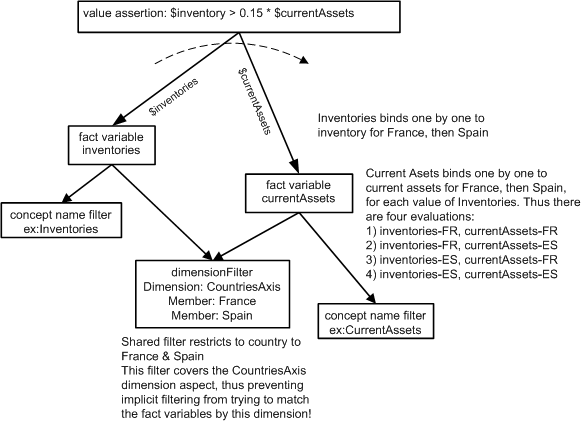Four evaluation results because the logic (as shown in the diagram) is to each of the two variables for each possible country, including the cross products, because the filter's dimension aspect is covered, preventing implicit filtering on dimension:

`Value Assertion assertion evaluations : 3 satisfied, 1 not satisfied`

### 7.3.5 Testing France dimension members inventory test (two dimension members on group filter)

This assertion moves the dimension filter to the assertion, where it is called a group filter, instead of a fact variable filter. The model of the assertion is in Figure 22. With use of the group filter, its aspect (CountriesAxis dimension) is not covered, therefore the formula processor can implicitly filter the fact variables to match the CountriesAxis dimension aspect of each other.

Figure 22: Assertion to test Assets to LiabilitiesAndEquity in each dimension by a group filter.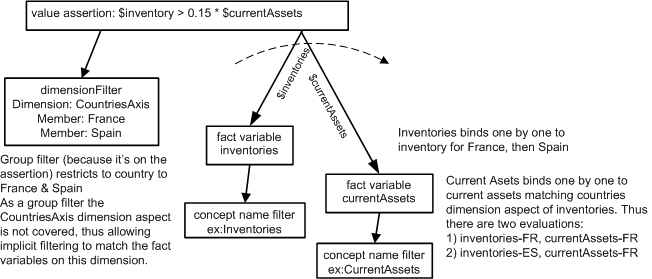Two evaluation results because the dimension filter has been moved to the assertion (so it is a group filter), which now leaves the CountriesAxis dimension uncovered, so the fact variables are implicitly matched by the countries dimension:

`Value Assertion assertion evaluations : 1 satisfied, 1 not satisfied`

# 8 Aspect models

An aspect is information about a XBRL instance fact that is in addition to its value, such as it concept, period, or dimensions. The aspects of a fact form the basis for matching and filtering facts. The aspects that apply to a fact and description of how that information is split into different aspects form an aspect model. XBRL formula defines two aspect models, dimensional and non-dimensional in a manner that allows for future extension models. Table 1 shows which aspects are present in the dimensional and non-dimensional aspect models for tuples, numeric items, and non-numeric items.

Table 1: Aspect models
Aspect Aspect model
Name Examples Dimensional Non-dimensional
Tuple Item Tuple Item
Numeric Non-numeric Numeric Non-numeric
Location Nesting within tuples (if applicable)
Concept Element name, type, substitution group
Entity identifier Scheme and identifier, values or patterns
Period Start, end, instant dates, or forever
Unit Single measure or multiply and divide measures
Complete segment XML fragment(s)
Complete scenario XML fragment(s)
Non-XDT segment XML fragment(s)
Non-XDT scenario XML fragment(s)
Dimension Explicit and typed dimensions including defaults

Aspects form the basis for matching and filtering facts. Filtering can be explicit or implicit. Explicit filtering can occur for the group of fact variables that apply to an assertion or other variable set, or for specific fact variables. For example, a group filter may restrict data to a specific period or dimension value, whereas a fact variable filter may bind a fact variable to a certain concept element name, or relate it to a period (such as filtering a balance for the start or ending of a changes duration period). Implicit filtering can match the aspects not otherwise covered (e.g. excluding concept name, but matching dates, dimensions, entity and units as applicable).

Each aspect has a specific matching test implied by the aspect. Concept aspects match by QName of the element, periods by their dates, entity identifiers by their scheme and value, units by their measures, dimensions (if dimensional) by their explicit members and typed contents, and segment and scenario by XML contents, called Open Context Components, or OCCs.

## 8.1 Custom aspect-matching tests

For the case of typed dimension aspects, a custom matching test can be supplied by user XPath expressions. This can be helpful when a typed dimension represents an XML structure that needs an XPath 2 expression to compare to another value of the same typed dimension for matching purposes.

# 9 Explicit filters

An explicit filter is one that is declared by a formula linkbase filter resource. It has a group filtering behaviour when related to a variable set (value assertion, existence assertion, or formula value and rules producing an output fact). Conversely, it has a fact variable filtering behaviour, when related to a fact variable. The same filter may be related to multiple fact variables and even have group behaviour in one variable set (assertion) and fact filtering behaviour in another variable set.

Filters may be marked as complement, meaning that their boolean result is inverted.

Filters may be combined by and and or relationships using boolean filters, which serve to build groups of filter terms much as parentheses group terms of an expression in a programming language.

Table 2 is a listing of filters by the filter name, aspects covered, filter options, and filter use. The order is by category of filtering action.

Table 2: Filters
Basis Specification Aspect coverable Filter Filter by
Fact aspect Concept Concept Name QName of fact element. May have multiple QName choices or an expression.
Period Type Concept schema declared period type, instant or duration.
Balance Concept schema declared balance, credit or debit.
Custom attribute Concept schema element declaration custom attribute value.
Data Type Concept schema declared data type.
Substitution group Concept schema declared substitution group.
Dimension Specific dimension Explict Dimension Options to select by presence of dimension, specific member of dimension, or axis relationship (child, descendant, etc) to specified member.
Typed Dimension Match by an XPath 2 test expression on the typed dimension.
Entity Entity identifier Identifier An XPath 2 test expression on the entity identifier.
Specific scheme Value to match
Regular expression scheme Scheme regular expression
Specific entity identifier Value to match
Regular expression entity identifier Entity identifier regular expression
General (none) General An XPath 2 test expression on a fact.
Period Period Period An XPath 2 test expression on the period.
Period Start Date and time values to match start
Period End Date and time values to match end
Period Instant Date and time values to match instant
Forever Filters facts with forever periods
Instant Duration Matches instant facts to start or end of another variable's duration fact.
Segment and Scenario Complete Segment Segment An XPath 2 test expression on the segment.
Complete Scenario Scenario An XPath 2 test expression on the scenario.
Tuple Location Parent QName of fact's parent element. May have QName or an expression.
Ancestor QName of any ancestor element of fact. May have QName or an expression.
Sibling Matches facts that are siblings of another variable's fact.
Location Matches facts that are related to another variable's fact by an XPath 2 relative path.
Unit Unit Single Measure QName of fact's unit measure. May have QName or an expression.
General Measures An XPath 2 test expression on the unit.
Value (none) Nil Matches facts reported as nil.
Precision An XPath 2 expression for minimum reported or inferred precision.
Boolean Logic Boolean (none) And And's result of related subfilters.
Or Or's result of related subfilters.
DTS Relationship Concept Relationship Concept Concept Relationship Matches facts of a concept that has a specified axis relationship to another variable in designated base set.
Coverage Aspect Cover as requested Aspect Cover Covers the requested aspects without any filtering of that aspect.
Match variable Match Concept Concept Match concept to another variable's bound fact.
Location Location Match location to another variable's bound fact.
Unit Unit Match unit to another variable's bound fact.
Entity Identifier Entity Identifier Match entity identifier to another variable's bound fact.
Period Period Match period to another variable's bound fact.
Dimension Specified dimension Match specified dimension to another variable's bound fact.
Complete Segment Complete Segment Match complete segment to another variable's bound fact.
Non-XDT Segment Non-XDT Segment Match non-XDT segment to another variable's bound fact.
Complete Scenario Complete Scenario Match complete scenario to another variable's bound fact.
Non-XDT Scenario Non-XDT Scenario Match non-XDT scenario to another variable's bound fact.
Relative Filter Relative All uncovered Relative Alternative to implicit filtering, matches all uncovered aspects to another variable's bound fact, and may cover those aspects.

## 9.1 Group filters (variable set filters)

As a group filter filters (constrains) all of the facts that the fact variables of the variable set may bind to. As a group filter, there is no interaction between the aspect covered by the filter and implicit filtering of the facts being evaluated by a variable set. A group filter does not cover any aspects, and thus the aspects which would be covered if the filter were a variable filter, are subject to implicit filtering or use by a relative filter.

## 9.2 Variable filters (also used in rendering)

As a fact variable filter, the aspect of a subject filter constrains facts for the fact variable(s) that it is related to.

### 9.2.1 Covering

Fact variable filters may cover aspects, which then excludes those covered aspects from implicit filtering of the related fact variable or matching by a relative filter. (Covering is not applicable to group filtering.)

# 10 Implicit filtering

Implicit filtering is used to match the facts bound to a variable set's fact variables in each of the uncovered aspects that each variable's fact(s) has(have), given the aspect model in effect. Implicit filtering doesn't do any matching on any aspect that is covered (when comparing any two facts by any aspect covered on any one of the twofacts).

Implicit filtering can be disabled, for a variable set, by an attribute on the variable set. When disabled, only explicit filtering and user XPath expressions will restrict input instance facts that may be bound to fact variables.

A model-view showing how implicit filtering operates uses the example of Figure 15, where there is a changes variable, filtered only by its concept name, to which balance variables are matched, after explicit filtering on concept name and instant duration period. The balance variables are in all other aspects matched to the concept fact.

The initial fact variable to be bound to a fact is \$changes, because both the balance variables depend on it (for explicit filtering of their respective periods). Figure 23 shows that initially all implicit search aspects are unconstrained. In the second column, a concept filter introduces a covered aspect, which is the concept aspect. (The concept name is not to be compared to other fact variables, so it is 'covered'.) In the third column, the aspects that may be carried forward, figuratively, to the next fact variable binding step, include unconstrained aspects for all but concept, which is 'changes'. When matched to a concept fact, as indicated in the rightmost column, the aspects carried forward to the next binding step reflect all the aspects of the \$changes fact (with concept name covered).

Figure 23: Implicit filtering, fact variable \$changes.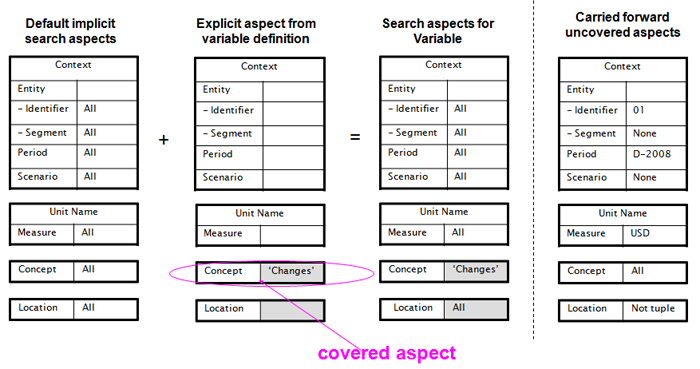The next fact variable to be bound to a fact is (either of) the balances fact variables. For this illustration we assume the starting balance is bound first, as shown in Figure 24. The search criteria for a starting balance begins with the aspects uncovered from \$changes, and an explicit filter for period (to match the start of the duration period of \$changes). The resulting search criteria are shown in the third column, with the bound fact's aspects in the right column.

Figure 24: Implicit filtering, fact variable \$beginningBalance.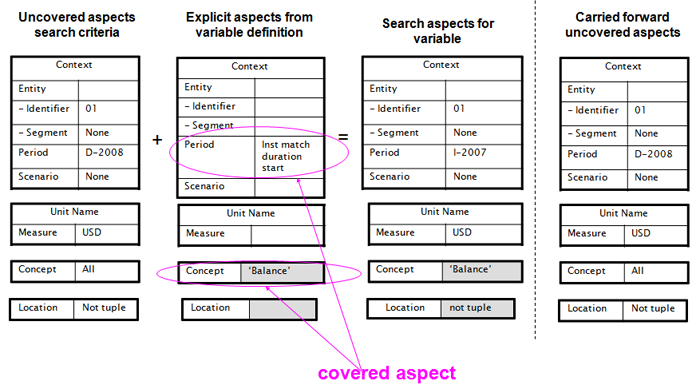The last fact variable to be bound to a fact is the other balances fact variable, as shown in Figure 25.

These figures are only used as an illustration, and belittle the actual logic used in explicit and implicit filtering. The trace steps of the original example, and corresponding source code of the open source project used to obtain the trace, provide one possible implementation strategy (but are not proscriptive of an expected strategy).

Figure 25: Implicit filtering, fact variable \$endingBalance.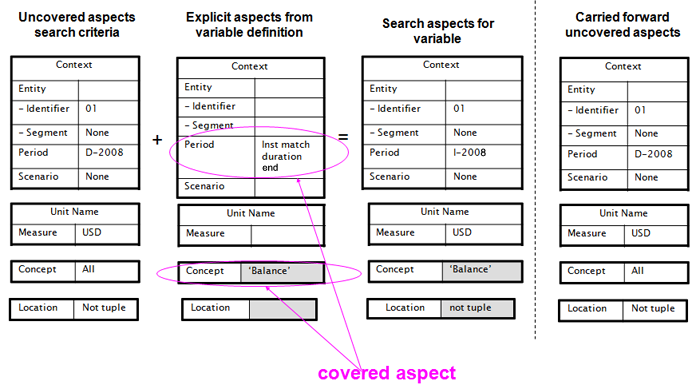# 11 Fact variable fallback values

The example of Section 7.3.2 introduced the notion of fallback values, which allowed the example to evaluate an an assertion which otherwise would not have evaluated because one of the facts was missing. A fallback value is useful to allow assertions and formulas to proceed to evaluation under these circumstances.

Fallback values are assigned to fact and general variables that have ``` @fallbackValue``` attributes when the variable binds to an empty sequence. For fact variables this means that no facts passing explicit and implicit filtering were identified.

A fallback value isn't helpful for the situation where all variables might fall back in the same evaluation, because for an evaluation (that has variaables) to proceed at least one variable must be bound to a nonempty sequence.

A fallback value is never assigned when to a fact variable that has a bound value in another like evaluation. This prevents having an evaluation where a fact is found duplicate an almost-clone evaluation where the same fact variable is assigned the fallback value. Logic needed to implement this is similar to other predicate-logic computer science languages that maintain a trail of 'visited' evaluations.

The expression for a fallback value cannot have a reference to another fact or general variable (but it may refer to a parameter). The reason it cant reference other variables is that would introduce a specified dependency order, where the desire for fallback values is to be able to substitute for any missing fact.

Fallback values for fact variables are always atomic (scalar valued, not node-valued). The fallback value has no aspects and does not participate in implicit filtering of other variables (and also would be ineffectual if used as an aspect reference variable for a relative filter, match filter, or dimension filter.

# 12 Existence assertions

An existence assertion is useful for checks of static existence, such as to assure that document descriptive facts such as form type, company identification, and filing identification are present. It is not useful for dependent checks of fact presence for dimension, period, and other reference qualifications, because the existence filter only operates globally. (A value assertion can be used in those cases.) The processing model of an existence figure is shown in Figure 26. The test expression of an existence assertion checks the count of completed evaluations of the variable set, but does not have access to any fact or general values of the completed individual evaluations.

Figure 26: Existence assertion processing model.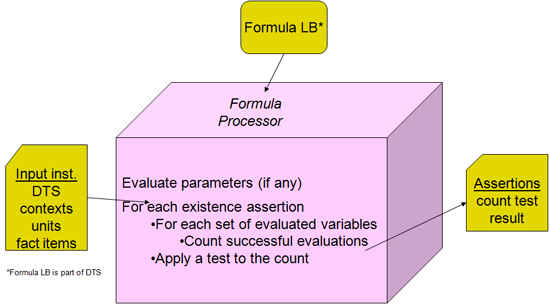## 12.1 Example testing existence of a net incomes fact

The first example is a companion to the example in Section 7.1, here testing that a net incomes fact has been reported. A tool-view of the corresponding assertion is shown in Figure 27. The top line is the existence assertion, with no test expression provided. This then will be successful if at least one evaluation of the existence assertion's variable set occurs (which is the case with the sample data in formula example 20). The model for how the assertion declares this is shown in Figure 28

Figure 27: Assertion to test existence of a net incomes fact.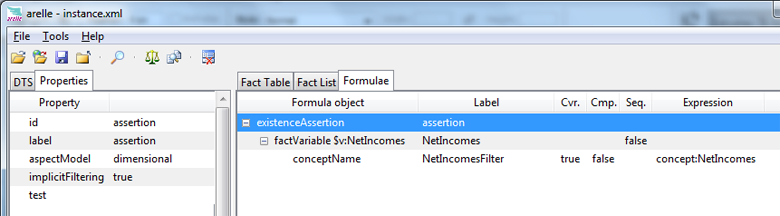A model-view of an existence assertion, based on the use of UML state symbols expressing a stateful outer counter for an inner state representing evaluation(s) of the variable set .

Figure 28: Existence assertion model.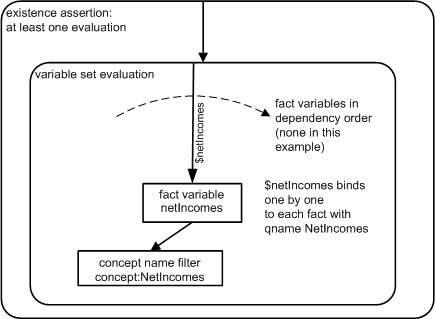# 13 Formulae to produce output XBRL instance fact items

Formulae are constructs in a formula linkbase that cause production of fact items. (Tuples can also be produced - see Section 19).

A formula (construct) is a variable-set that causes an output fact item to be produced for each evaluation of the variable set. The fact item's value is specified by an XPath expression that can (and usually does) refer to the bound variables of the evaluation, and has rules that specify a numeric fact's accuracy, and the values for each aspect of the output fact (concept, period, entity identifier, unit for numerics, and dimensions or segment/scenario as applicable). The aspect rules provide a spectrum of capability, from the simple reuse of aspects of bound fact variables, to an ability to construct each aspect by declarative rules.

Fact items are produced into a standard output XBRL instance, or may be directed into specified output XBRL instances (see Section 18), such as to create more than one output XBRL instance. The output XBRL instances may be stored in files or used in chaining (see Section 18.1).

Fact items produced by a formula construct can be compared to fact items expected in an input XBRL instance, comparing values against tolerance margins using consistency assertions (see Section 14). Whereas a value assertion provides a filter-based mechanism to assert something about an input fact, the use of a formula with a consistency assertion provides an aspect rules based fact construct production match. Facts produced for the purpose of consistency-assertion matching to input facts exist in processor memory for the comparison process (according to the application implementation). In addition they may also be output to an XBRL instance file, and may be provided by chaining to dependent variable sets.

The model for formula fact production is shown in Figure 29. This model begins in the same manner as that of value assertions, but where the value assertion would be evaluated, the value and output fact are produced. The common actions are to obtain values for parameters, bind the variables representing the terms to be evaluated to input XBRL instance facts, according to filtering in effect, and test the precondition. The difference is that the formula must next evaluate the rules that specify value, decimals or precision, and aspects, in order to complete the fact item for the output XBRL instance.

Figure 29: Fact production processing model.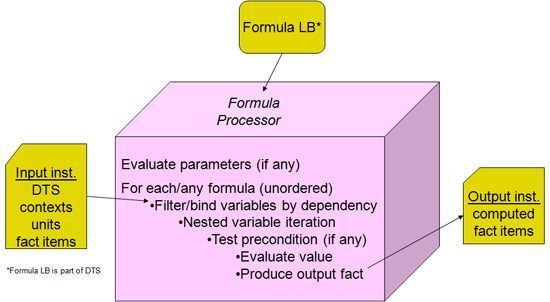## 13.1 Value Rules

The value rule is an XPath expression that yields the value to be assigned to the fact. It can be a simple expression, such as a constant, or it can contain terms which refer to variables and parameters of the variable set, chained values from other variable sets, and computed values from custom and built-in functions. A xsi:nil value can be produced by assigning an empty sequence to the value.

Example 10: Formula value example linkbase syntax

``` <formula:formula xlink:type="resource" xlink:label="formula1" value="1.2" source="total" aspectModel="dimensional" implicitFiltering="true"><formula:decimals>1</formula:decimals></formula:formula> ``` A numeric fact item has the value 1.2, accuracy specified as decimals=1, and all the aspects are copied from another variable (\$total), including the concept name, periods, entity, unit, and any dimensions.
``` <formula:formula xlink:type="resource" xlink:label="formula1" value="'hello world'" source="formula:uncovered" aspectModel="dimensional" implicitFiltering="true"/> ``` A string fact item is assigned a string constant, which must be enclosed in quotes for XPath syntax to recognize it as a string context within an XPath expression. As this item is a string, no accuracy rule applies. The aspects are taken from any any variable where the aspect is uncovered.
``` <formula:formula xlink:type="resource" xlink:label="formula1" value="sum(\$children)" source="children" aspectModel="dimensional" implicitFiltering="true"><formula:decimals>2</formula:decimals></formula:formula> ``` A sequence of children are summed and written to a total. The accuracy is specified for two decimals. The aspects including concept name are copied from \$children (which means that the output fact will have the same concept name, period, entity, and dimensions).

### 13.1.1 Accuracy Rules

A formula which produces non-fraction numeric items may have an accuracy rule, to specify decimals or precision of the fact. If the accuracy rule is omitted, the fact is generated with precision zero, specifying that "nothing is known about its accuracy". The accuracy rule, itself an XPath expression, may provide a constant (such as `decimals="2"`) or provide accuracy in another way (such as to copy it from a fact, e.g. `\$total/@decimals`).

## 13.2 Aspect Rules

Aspect rules are nested XML constructs in the formula:formula (or tuple:tuple) construct. (This differs from fact variable filters which are relationship-connected to fact variables.) Aspects may be specified by rule or source, or may be defaulted. Except for the `formula:location` aspect, which is always defaulted, the other aspects have a default only if they have a source (a rule that specifies how to get the aspect from a bound variable of the evaluation). Some aspects are combinable, such as dimensions, segment and scenario fragments, and units, because they can accumulate terms from possibly multiple sources and/or specific rules.

Table 3: Aspect rules
Aspect Rule
Name Description Combinable
Formula Item Rules
formula:location This rule never appears in a formula, its default always specifies a root-located fact.
formula:concept Element name, inherited from nearest source or explicitly specified by QName or QName expression
formula:entityIdentifier Scheme and identifier may be inherited from nearest source or explicitly specified by rule.
formula:period Start, end, instant dates, or forever may be inherited from nearest source or explicitly specified by rule.
formula:explicitDimension Explicit and typed dimension values may be inherited from nearest source or explicitly specified by rule. Dimension terms are combinable.
formula:typedDimension
formula:occFragments XML fragment(s) comprising segment and scenario.
formula:occXpath
formula:unit Unit measure, numerator, and divisor, values may be inherited from nearest source or explicitly specified by rule. Multiply and divide terms are combinable.
Formula Tuples Rules
tuple:location Extension to specify location within a tuple. May be explicit rule or inherited source, usually via variables-scope chaining. Vaue of the parent (tuple) of the item/tuple being produced.

### 13.2.1 Source

An aspect may be obtained (in part or full) from a bound variable of the evaluation, by specifying a source. Source may be specified on a rule, and may be inherited from a source on the formula (or tuple) element. When there are multiple sources, the nearest one to an aspect rule prevails.

Example 11: Nearest sources

<formula:aspects>
<formula:entityIdentifier value="'ABCD-1234'"/>
</formula:aspects>
<formula:aspects source="eg:variableB">
<formula:period/>
<formula:unit source="eg:variableC">
<formula:multiplyBy source="eg:variableD"/>
</formula:unit>
</formula:aspects>
</formula:formula>
aspect rule nearest source
entity identifier `eg:variableA`
period `eg:variableB`
unit `eg:variableC`
unit multiplication `eg:variableD`

A formula source may either ba a QName of a variable, or `formula:uncovered`. Specifying `formula:uncovered` directs the processor to obtain the aspect variable from any of the variable set's bound factVariables that have the aspect uncovered (because implicit filtering forces all variables of the evaluation to be matched by the uncovered aspect values),

### 13.2.2 Non-combinable Rules

Aspects that have a single value for a fact are non-combinable. These include location, concept, entity identifier, and period.

The location rule can only be specified for tuple-parented facts, with the `tuple:location` rule. See Section 19. The location rule will always use a ``` @source``` to refer to the variable bound (by variables-scope chaining) to the tuple that is to be the parent.

The concept rule us usually needed when the output fact is for a different concept than the variables of the terms, such as producing a total = sum( \$parts ) or c = \$a + \$b.

Example 12: Concept rules

<formula:decimals>
0
</formula:decimals>
<formula:aspects>
<formula:concept>
<formula:qname>
eg:total
</formula:qname>
</formula:concept>
</formula:aspects>
</formula:formula>
The formula's result is a concept eg:total, specified as a QName constant
<formula:decimals>
2
</formula:decimals>
<formula:aspects>
<formula:concept>
<formula:qnameExpression>
node-name(\$c)
</formula:qnameExpression>
</formula:concept>
</formula:aspects>
</formula:formula>
The formula's result is a concept that dynamically takes the QName of the fact that \$c is bound to.

### 13.2.3 Combinable Rules

Aspects that have a multiple values for a fact are combinable. These include dimensions, segment and scenario rules, and units. Combinable rules may begin by copying aspect value(s) from a source (bound fact variable), and may add additional aspects by their rule (such as adding multiple multiplyBy and divideBy measures to a unit aspect). They may also begin by canceling out (omitting) specified combinable aspect values inherited from their nearest source.

In the case of combinable values for units, the multiplyBy and divideBy measures are normalized, by cancelling out any measures that occur in both the multiplyBy and divideBy measures.

# 14 Consistency assertions

A consistency assertion specifies how to determine whether an output fact, produced by the associated formula, is consistent with all aspect matched facts in the input XBRL instance. For example, if a formula \$c = \$a + \$b produced an output fact item eg:c, its consistency assertion could state that the output eg:c must be within a tolerance margin, called acceptance radius, of the value of a corresponding input fact (with the same aspects, e.g., same concept name, period, entity identifier, unit, and dimensions).

For numeric facts, the acceptance radius can be specified as a proportion (such as within 0.05 representing 5%), or an absolute value (such as within \$5.00, if the units were USD).

For the case where there are multiple aspect-matched facts in the input instance, they must all be consistent for the consistency evaluation to succeed. Any inconsistency makes the evaluation report being not-successful.

An attribute, ``` @strict```, specifies whether the consistency assertion is evaluated for produced output facts when there is no matching input fact. If strict is true and there is no matching input fact, the assertion fails if the produced fact value is non-nil (or succeeds when the produced fact value is nil). If strict is false then the consistency assertion isn't evaluated when there are no input facts, which could mean that the user does not get a report whether consistency was true or false, it just isn't evaluated.

Figure 30: Consistency assertion processing model.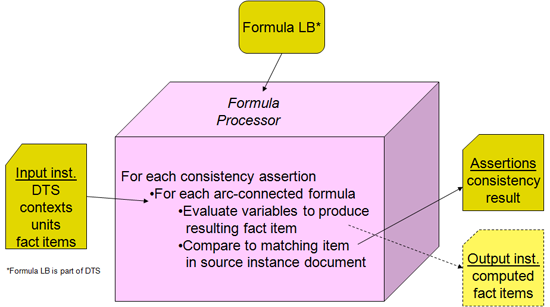# 15 Validation messages

The purpose of validation messages is to enhance the user friendliness of the reports by a formula processor. The messages are composed by incorporating variable and aspect values into message text, such as to provide feedback like: “Your submission of form {\$formType} is missing a {\$omission} for context {\$formType/@contextRef}”.

Validation messages allow associating messages to an assertion, to compose text (or structured XML) for successful or not-successful evaluations. For case (success or not) there may be multiple messages in different languages (if coding the alternate languages in the linkbase is chosen by the application strategy).

An assertion is connected to its messages by relationships with an arcrole identifying whether the message is associated with assertion success, or the case of the assertion unsuccessful. An implementation may chose to report the message to its user interface, logging system, or other means, to convey to the user assertion results.

The message can embed XPath 2 expressions in the text to compose meaningful messages relating to the corresponding evaluation. For a value and consistency assertion, the XPath expression has access to the variables of the evaluation and parameters. For an existence assertion, the assertion is only fired after all existence counts have been evaluated, so no variable-set variables are accessible to the XPath expression.

The assertion messages can be designed to apply to multiple assertions, by use of the XPath expressions to identify what facts and situations are being reported.

Example 13: Validation messages

Not satisfied error: Fact { node-name(\$var) } in context { \$var/@contextRef }, reported value { \$var }, for the period starting { xs:date( xfi:period-start( xfi:period(\$var) ) ) } and ending { xs:date( xfi:period-end( xfi:period(\$var) ) - xs:dayTimeDuration('P1D') ) }
</msg:message>
This message uses XPath expressions to produce a text message such as "Not satisfied error: Fact tax:grossProfits in context D-2008-Totals, reported value 1234.56, for the period starting 2010-01-01 and ending 2010-12-31. The period dates are reported without the time part (the xfi:period function provides a date-time, but the users expect the date without time). The ending date, which would be the following midnight (e.g., 2011-01-01T00:00:00) has to be made one day earlier when stripping the time portion. In this case the message is "hard-wired" to a specific variable, \$var.
Not satisfied error: Fact { xff:uncovered-aspect('concept') }, for the period starting { xs:date( xfi:period-start( xff:uncovered-aspect('period') ) ) } and ending { xs:date( xfi:period-end( xff:uncovered-aspect('period')) ) - xs:dayTimeDuration('P1D') }
</msg:message>
The use of functions to obtain contextual data is shown. The uncovered-aspect function allows composing the message without being dependent on the variable name in an assertion, for example if one message is shared among multiple assertions that have different variable terms. In this case the uncovered-aspect function provides the concept name, period start, and period end, based on the implicit filtering uncovered aspects of the evaluation.

# 16 Function registry overview

The functions registry provides standard functions implemented within a formula processor.

Table 4: Registry functions
Registry Sequence Signature Description
80101 xfi:context(schema-element(xbrli:item)) Returns the item's context element: element(xbrli:context)
80102 xfi:unit(schema-element(xbrli:item)) Returns the item's unit, if any: element(xbrli:unit)?
80103 xfi:unit-numerator(element(xbrli:unit)) Returns unit numerator measures: element(xbrli:measure)+
80104 xfi:unit-denominator(element(xbrli:unit)) Returns unit denominator measures: element(xbrli:measure)*
80105 xfi:measure-name(element(xbrli:measure)) Returns the QName of a measure element
80120 xfi:period(schema-element(xbrli:item)) Returns the period element of an itme: element(xbrli:period)
80121 xfi:context-period(element(xbrli:context)) Returns the period element of a context element: element(xbrli:period)
80122 xfi:is-start-end-period(element(xbrli:period)) True if period is a start-end period.
80123 xfi:is-forever-period(element(xbrli:period)) True if period is a forever period.
80124 xfi:is-duration-period(element(xbrli:period)) True if period is a duration period (either start-end or forever).
80125 xfi:is-instant-period(element(xbrli:period)) True if period is an instanct period.
80126 xfi:period-start(element(xbrli:period)) Teturns period's start dateTime.
80127 xfi:period-end(element(xbrli:period)) Returns period ending (or instant) dateTime. This is the following midnight if a date is reported without time.
80129 xfi:period-instant(element(xbrli:period)) Returns instant dateTime. This is the following midnight if a date is reported without time.
80130 xfi:entity(schema-element(xbrli:item)) Returns the entity element of the context of an item.
80131 xfi:context-entity(element(xbrli:context)) Returns the entity element of a context.
80132 xfi:identifier(schema-element(xbrli:item)) Returns the identifier element of the context of an item.
80133 xfi:context-identifier(element(xbrli:context)) Returns the identifier element of a context.
80134 xfi:entity-identifier(element(xbrli:entity)) Returns the identifier element of an entity.
80135 xfi:identifier-value(element(xbrli:identifier)) Returns the identifier value of an identifier element.
80136 xfi:identifier-scheme(element(xbrli:identifier)) Returns the scheme of an identifier element.
80137 xfi:segment(schema-element(xbrli:item)) Returns the segment element of the context of an item, if any.
80138 xfi:entity-segment(element(xbrli:entity)) Returns the segment element of an entity element, if any.
80139 xfi:context-segment(element(xbrli:context)) Returns the segment element of a context element, if any.
80140 xfi:scenario(schema-element(xbrli:item)) Returns the scenario element of the context of an item, if any.
80141 xfi:context-scenario(element(xbrli:context)) Returns the scenario element of a context element, if any.
80142 xfi:fact-identifier-value(schema-element(xbrli:item)) Returns the entity identifier value of the context of an item.
80143 xfi:fact-identifier-scheme(schema-element(xbrli:item)) Returns the entity identifier scheme of the context of an item.
80150 xfi:is-non-numeric(xs:QName) Returns true if QName is a non-numeric concept item, and neither a tuple nor fractional item.
80151 xfi:is-numeric(xs:QName) Returns true if QName is a numeric concept item (including if fraction) and not a tuple.
80152 xfi:is-fraction(xs:QName) Returns true if QName is a fraction numeric item.
80153 xfi:precision(schema-element(xbrli:item)) Return the actual or the inferred precision of a numeric fact item. Raises an exception if not a numeric fact item.
80154 xfi:decimals(schema-element(xbrli:item)) Return the actual or the inferred decimals of a numeric fact item. Raises an exception if not a numeric fact item.
80155 xff:uncovered-aspect(xs:token, xs:QName?) Returns the specified uncovered aspect for use in XPath expressions of a consistency assertion, value assertion, formula aspect rule, or generic message XPath expression. The function is not applicable to variable-set variable evaluation and filter expressions.
80156 xff:has-fallback-value(xs:QName) Returns true() for factVariables that have been assigned a fallback value, for use in XPath expressions of a precondition, a consistency assertion, value assertion, formula aspect rule, or generic message XPath expression. The function is not applicable to variable-set variable and filter expressions.
80157 xff:uncovered-non-dimensional-aspects() Returns a sequence containing the set of the uncovered non-dimensional aspects for use in XPath expressions of a consistency assertion, value assertion, formula aspect rule, or generic message XPath expression. The function is not applicable to variable-set variable evaluation and filter expressions.
80158 xff:uncovered-dimensional-aspects() Returns a sequence containing the set of the uncovered dimensional aspects for use in XPath expressions of a consistency assertion, value assertion, formula aspect rule, or generic message XPath expression. The function is not applicable to variable-set variable evaluation and filter expressions.
80200 xfi:identical-nodes(node()*, node()*) Returns true if the identical node comparison defined in the XBRL 2.1 specification is true for the two sequences of nodes supplied as arguments.
80201 xfi:s-equal(node()*, node()*) Returns true if two node sequences are s-equal.
80202 xfi:u-equal(node()*, node()*) Returns true if two item sequences are u-equal.
80203 xfi:v-equal(node()*, node()*) Returns true if two item sequences are v-equal.
80204 xfi:c-equal(node()*, node()*) Returns true if two item sequences are c-equal.
80205 xfi:identical-node-set(node()*, node()*) Returns true if for every node in the left sequence there is an identical node in the right sequence, and the sequences have the same count of members.
80206 xfi:s-equal-set(node()*, node()*) Returns true if for every node in the left sequence there is an s-equal node in the right sequence, and the sequences have the same count of members.
80207 xfi:v-equal-set(node()*, node()*) Returns true if for every item in the left sequence there is a v-equal item in the right sequence, and the sequences have the same count of members.
80208 xfi:c-equal-set(node()*, node()*) Returns true if for every item in the left sequence there is a c-equal item in the right sequence, and the sequences have the same count of members.
80209 xfi:u-equal-set(node()*, node()*) Returns true if for every item in the left sequence there is a u-equal item in the right sequence, and the sequences have the same count of members.
80210 xfi:x-equal(node()*, node()*) Returns true if two node sequences are x-equal.
80211 xfi:duplicate-item(schema-element(xbrli:item), schema-element(xbrli:item)) Returns true if two items are duplicates.
80212 xfi:duplicate-tuple(schema-element(xbrli:tuple), schema-element(xbrli:tuple)) Returns true if two tuples are duplicates.
80213 xfi:p-equal(element()+, element()+) Returns true if two nodes (each an item or tuple) are children of the identical parent.
80214 xfi:cu-equal(node()*, node()*) Returns true if two sequences have items that are both c-equal and u-equal.
80215 xfi:pc-equal(node()*, node()*) Returns true if two sequences have items that are both c-equal and p-equal.
80216 xfi:pcu-equal(node()*, node()*) Returns true if two sequences have items that are both c-equal, u-equal, and p-equal.
80217 xfi:start-equal(xbrldi:dateUnion, xbrldi:dateUnion) Returns true if two arguments are equal in period start dateTime. Each argument may be either a xs:date or an xs:dateTime (e.g., xbrli:dateUnion). If arguments are mixed (one xs:date and other xs:dateTime) the xs:date is defined as the xs:dateTime of the midnight starting the date (00:00 hours of that date).
80218 xfi:end-equal(xbrldi:dateUnion, xbrldi:dateUnion) Returns true if two arguments are equal in period end or instant dateTime. Each argument may be either a xs:date or an xs:dateTime (e.g., xbrli:dateUnion). If arguments are mixed (one xs:date and other xs:dateTime) the xs:date is defined as the xs:dateTime of the midnight ending the date (24:00 hours of that date).
80219 xfi:nodes-correspond(node(), node()) Returns true if both if and only if the two argument nodes are both attribute nodes that correspond or both element nodes that correspond. It returns a boolean value of false otherwise. Compares atomized non-id attributes, in any order, and atomized elements in their order, using the XPath 2.0 eq operator.
90101 xfi:facts-in-instance(element(xbrli:xbrl)) Obtains a sequence of all facts in an XBRL instance.
90102 xfi:items-in-instance(element(xbrli:xbrl)) Returns the sequence of all fact items that are direct children of the root element of an XBRL instance document (excluding any items nested in tuples). The data type of each fact item has the appropriate type based on the Post Schema Validation Infoset.
90103 xfi:tuples-in-instance(element(xbrli:xbrl)) Returns the sequence of all tuples that are direct children of the root element of an XBRL instance document (excluding any tuples nested in tuples).
90104 xfi:items-in-tuple(element(xbrli:tuple)) Returns the sequence of all fact items that are direct children of the tuple element of an XBRL instance document (excluding any items further nested in enclosed tuples).
90105 xfi:tuples-in-tuple(element(xbrli:tuple)) Returns the sequence of all tuples that are direct children of the tuple element of an XBRL instance document (excluding any tuples further nested in enclosed tuples).
90106 xfi:non-nil-facts-in-instance(element(xbrli:xbrl)) Obtains a sequence of all the facts that do not have nil values in an XBRL instance.
90201 xfi:concept-balance(xs:QName) Obtain the value of the xbrli:balance attribute on an XBRL concept.
90202 xfi:concept-period-type(xs:QName) Obtain the value of the xbrli:periodType attribute on an XBRL concept given the QName of the XBRL concept as input.
90203 xfi:concept-custom-attribute(xs:QName, xs:QName) Obtain the value of of an attribute on an XBRL concept declaration that is not in the XBRL instance or XML Schema namespaces.
90204 xfi:concept-data-type(xs:QName) Obtain the QName of the data type of an XBRL concept.
90205 xfi:concept-data-type-derived-from(xs:QName, xs:QName) Tests whether one the XML Schema data type of a concept is derived from another XML Schema data type.
90206 xfi:concept-substitutions(xs:QName) Obtains an ordered sequence of QNames of the elements that the concept is in the substitution group for.
90213 xfi:filter-member-network-selection(xs:QName, xs:QName, xs:string, xs:string, xs:string) Returns a sequence containing a select set of dimension member QNames for the specified explicit dimension considering only those members that have the specified relationship axis to the specified origin member in the network of effective relationships with the specified link role for the specified arc role. The set of dimension member QNames is in an arbitrary order (not necessarily that of effective tree relationships order).
90214 xfi:filter-member-DRS-selection(xs:QName, xs:QName, xs:QName, xs:string?, xs:string) Returns a sequence containing a select set of dimension member QNames for the specified explicit dimension considering only those members that have the specified relationship axis to the specified origin member in the network of effective relationships with the specified link role for the specified arc role. The set of dimension member QNames is in an arbitrary order (not necessarily that of effective tree relationships order). Note that the relationships considered by this function are those expressed by an arc elements that conform to the requirements set out in the XBRL Dimensions specification.
90304 xfi:fact-segment-remainder(schema-element(xbrli:item)) Return the content of a segment that is not reporting a XBRL Dimensions Specification based dimension value.
90305 xfi:fact-scenario-remainder(schema-element(xbrli:item)) Return the content of a scenario that is not reporting a XBRL Dimensions Specification based dimension value.
90306 xfi:fact-has-explicit-dimension(schema-element(xbrli:item), xs:QName) Tests whether the fact reports a value for a dimension in either the segment or scenario of the supplied item.
90307 xfi:fact-has-typed-dimension(schema-element(xbrli:item), xs:QName) Tests whether the fact reports a value for a dimension in either the segment or scenario of the supplied item.
90308 xfi:fact-has-explicit-dimension-value(schema-element(xbrli:item), xs:QName, xs:QName) Tests whether the fact reports the member for a dimension in either the segment or scenario of the supplied item.
90309 xfi:fact-explicit-dimension-value(schema-element(xbrli:item), xs:QName) Returns the QName, if any, of the member reported for the dimension in either the segment or scenario of the supplied item.
90310 xfi:fact-typed-dimension-value(schema-element(xbrli:item), xs:QName) Returns the child element of the segment or scenario that contains the typed dimension value if there is a value for the dimension in either the segment or scenario of the item and returns the empty sequence otherwise.
90403 xfi:fact-dimension-s-equal2(schema-element(xbrli:item), schema-element(xbrli:item), xs:QName) Returns true if both items have the same value (default or explicit) for the specified dimension (regardless of whether in segment or scenario).
90501 xfi:linkbase-link-roles(xs:string, element(xbrli:xbrl)) Returns a sequence containing the set of extended link role URIs having arcs of the subject arc role URI.
90503 xfi:concept-label(xs:QName, xs:string?, xs:string?, xs:string, element(xbrli:xbrl)) Returns a string containing the label that has the specified link role, resource role, and language.
90504 xfi:arcrole-definition(xs:string, element(xbrli:xbrl)) Returns a string containing the definition of the arcrole, or an empty sequence if none.
90505 xfi:role-definition(xs:string, element(xbrli:xbrl)) Returns a string containing the definition of the role, or an empty sequence if none.
90506 xfi:fact-footnotes(element(), xs:string?, xs:string?, xs:string?, xs:string) Returns strings containing the footnotes that has the specified link role, resource role, and language.
90507 xfi:concept-relationships(xs:QName, xs:string?, xs:string, xs:string, xs:nonNegativeInteger?, xs:QName?, xs:QName?, element(xbrli:xbrl)) Returns a sequence containing the set of effective relationships with the specified relationship to the source concept. This is a sequence of effective relationships that are implementation-defined objects or relationship surrogates. These objects are opaque as they may be used only as function arguments, but not for direct XPath navigation or value access. The implementation-defined objects or relationship surrogates are intended to be only used as parameters to other functions such as xfi:relationship-from-concept, xfi:relationship-to-concept, xfi:relationship-attribute, xfi:relationship-element, xfi:link-attribute, and xfi:link-element.
90508 xfi:relationship-from-concept(xfi:relationship.type) Returns a QName of the from (origin) concept of an effective relationship.
90509 xfi:relationship-to-concept(xfi:relationship.type) Returns a QName of the to (destination) concept of an effective relationship.
90510 xfi:distinct-nonAbstract-parent-concepts(xs:string?, xs:string, element(xbrli:xbrl)) Returns a sequence of relationship parents that represent non-abstract concepts and have non-abstract children.
90511 xfi:relationship-attribute(xfi:relationship.type, xs:QName) Returns a typed (PSVI) value of the designated attribute of an effective relationship's arc.
90512 xfi:relationship-link-attribute(xfi:relationship.type, xs:QName) Returns a typed (PSVI) value of the designated attribute of an effective relationship's parent link element.
90513 xfi:relationship-name(xfi:relationship.type) Returns a QName of the arc element of an effective relationship's arc. May be helpful to designate base set when multiple arc elements can be used on an arcrole in same link element.
90514 xfi:relationship-link-name(xfi:relationship.type) Returns a QName of the link parent element of an effective relationship's arc. May be helpful to designate base set when multiple link elements can be used with an arcrole.
90601 xfi:format-number(numeric?, xs:string) Provides an implementation of the XSLT 2.0 format-number function (which is not part of XPath2 functions), in a manner that is compatible with XBRL processors.

# 17 Custom functions

Functions built-into formula processors include the full set of XML schema constructors, such as xs:time() and xs:QName, the functions built into XPath 2, such as node-name and index-of, and the functions that are part of the functions registry. In addition, custom functions can extend the the built-in function set.

Custom functions are declared by providing a global declaration of the function signature. The function is then usable in all the XPath expressions of formula linkbases, including parameters, variables, filters, and any other executable XPath expressions.

Custom functions may be implemented by the formula processor, such as by providing Java or Python code according to the implementation language of the formula processor, or by providing custom function implementations in the formula linkbase, using XPath custom function implementation steps. The linkbase implementations are portable among formula processor products, whereas Java or Python coded implementations are dependent on the interface and processor architecture of an individual product (but are likely to be faster in execution, and have more access to non-instance DTS objects).

## 17.1 Custom functions implemented within linkbase

Custom functions implemented in the linkbase as a sequence of XPath 2 expression steps. Each step results in a value that can be referenced by name in subsequent steps, providing a manner of structure in addtion to the expressions of XPath.

Expression steps are executed in document order. Functions may call other functions and may call themselves recursively.

Example 14: Custom function implementations

Explanation CFI's signature CFI's implementation
Trims leading and trailing spaces from the input string. `eg:trim (\$input as xs:string?) as xs:string`
<cfi:implementation>
<cfi:input name="arg"/>
<cfi:output>
replace(replace(\$arg,'\s+\$',''),'^\s+','')
</cfi:output>
</cfi:implementation>
Return true if and only if the input can be cast to a numeric type. `eg:isNumeric (\$input as xs:anyAtomicType?) as xs:boolean`
<cfi:implementation>
<cfi:input name="value"/>
<cfi:output>
string(number(\$value)) != 'NaN'
</cfi:output>
</cfi:implementation>
Formula to compute the present value of an amount: `\$amountDue * my-fn:power((1 + \$interestRate), \$numYears)` `my-fn:power(\$y as xs:decimal, \$exp as xs:decimal) as xs:decimal`
<!-- positive integer power function -->
<variable:input type="xs:decimal"/>
<variable:input type="xs:decimal"/>
</variable:function>
<!-- Arc from formula 1 to the implementation -->
<!-- positive integer power function -->
<cfi:input name="y"/>
<cfi:input name="exp"/>
<cfi:output>
if (\$exp lt 0) then ( 1 div my-fn:power(\$y, - \$exp) ) else ( if (\$exp lt 1) then 1 else (\$y * my-fn:power(\$y,\$exp - 1)) )
</cfi:output>
</cfi:implementation>

## 17.2 External programmed custom functions

External programmed custom functions rely on the implementation language of the formula processor, and the object model and API of its implementation. They thus may be highly efficient, for difficult computational situations, and will have access to the full object model of the formula processor. This may provide complete access to the object model of the DTS (schema files, and linkbases), and complete access to business systems of the processor environment (such as SQL databases, business warehouses, and business intelligence analytics).

# 18 Multi-instance overview

The requirements for multi-instance processing began with use cases and with the same technology fulfilled a need for formula chaining, where the output of one set of steps produces fact variables that can be consumed by subsequent processing steps.

Use cases for multi-instance are the need to process reports:

• Multiple companies reporting, such as in IFRS or US-GAAP, Cross Industries Reporting Analysis
• Different company extension taxonomies (such as U.S. SEC filers)
• Cross Border Reporting Analysis
• Public Company F.S.: EDINET (Japan) and US Gaap (USA)
• Private Company F.S.: Infogreffe (Fr), NBB (Belgium), Infocamere (It)…
• Multiple periods reporting
• Different taxonomy year, linkbases changed
• Multiple types of reports
• Different taxonomies for each data sources such as
• Statistics Bureau & Corporate Registry
• Stock Exchange & Statistics Bureau

The approach suggested in the early modules of formula linkbase was to merge the instance documents to a neutral taxonomy and consolidated instance before processing, as represented by Figure 31. A number of difficulties arise in trying to merge. Usually there are multiple quarters or years, with different taxonomies per year. One finds that Taxonomy namespaces often change, linkbases become incompatibly different, particularly in structure, and sub-trees that define reporting semantics (either in terms of totaling of presented concepts or dimension member aggregation) differ for each period. Developers were encouraged to find a multi-instnace solution that did not require this processing step.

Figure 31: Instance merging processing model.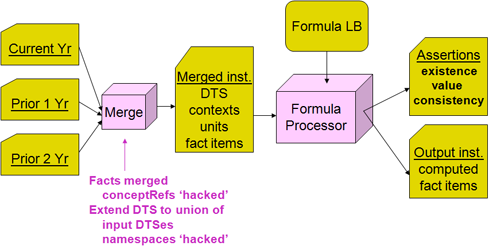Semantic issues with merging relate to concepts changing with changes in law, practice, dimensions changes, different tree relationships in presentation and dimensional definition, and altered contextRef’s.

The approach provided for multi-instance processing solves this by having each instance loaded to the formula processor with its own DTS intact, and not co-mingled with the schemas and linkbases of DTSes of other instances (insofar as they may be different). Figure Figure 32 shows this approach.

Figure 32: Separate multi-instances processing model.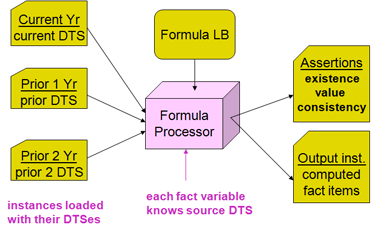In this approach chaining of formulas is also accomplished. This provides a common solution to multi-instance and chaining, wherein multi-instances act as ‘scratch-pads’ during computation (as described in Section 18.1.

Instances are represented by a resource, which is derived from parameter, so that it is accessible to the processor's invocation and external interface mechanism. An instance-variable relationship connects instance(es) to a variable (so that the variable knows to obtain its facts from the related instance(s) instead of the standard XBRL input instance. Likewise the output fact produced by a formula can be directed to a specific instance (other than the standard XBRL output instance) by a formula-instance relationship from the formula. The linkbase contents in this usage are represented as shown in Figure 33

Figure 33: Linkbase contents in multi-instance processing.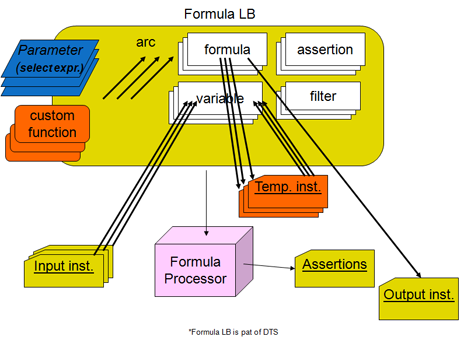There is no change to aspect processing with multi-instance implementation. Formula aspects come from its variables; variables from different instances each contribute aspects, but the aspects remain independent of the instances they come from. Aspect “covering” is by-aspect, not by-instance.

This example shows a simple test case

• There are three input instances, representing
• a current year,
• a prior year, and
• the second prior year
• Based on SVN test case dim-1-formula1.xml in directory 60300 instances-processing.
• The test case highlights the issue of having each of the 3 instances have different DTSes with the same namespace (in different child directories, of course!), where each year has different member hierarchies in the dimension members. Because the namespaces are the same, all aspects, including concept and dimension QNames can be matched up. (A real example would have to cover period, but this is just a minimaal example). The model of the example is in Figure 34. The result simply has a fact for each of the aspect matched input facts (of the 3 input instances) with the sum (across the instances).

Figure 34: Linkbase contents in multi-instance processing.Example 15: Summing aspect-matched facts across three input instances

Input instance B is the second input instance. The formula processor associates this instance with the second XBRL input instance file (in the test case, this is accomplished by the testcase XML file, but in a production environment, it would be by the application.
Input instance C is the third input instance. The formula processor associates this instance with the third XBRL input instance file.
<formula:decimals>
0
</formula:decimals>
</formula:formula>
The formula produces an output for each evaluation of implicitly-matched factVariable items from the three input instances (standard input instance, instance B, and instance C).
Fact variable inst-a-item bounds to facts from the standard input instance. The factVariable is the target of only one relationships, (1) variable-set, from the formula, and does not have an instance-variable relationship, as its source is the standard input instance.
Fact variable inst-b-item bounds to facts from input instance B. The factVariable is the target of two relationships, (1) instance-variable from instance B and (2) variable-set, from the formula.
Fact variable inst-c-item bounds to facts from input instance C.

## 18.1 Chaining by instance relationships

This example shows two formulas related by chaining, A = B + C, and C = D + E.

• Formula 1 (A=B+C): Result is A, factVariables B & C factVariable B is from source instance (default) factVariable C is from temp instance (has an arc)
• Formula 2 (C=D+E): Result is C, factVariables D & E, to temp instance factVariables D & E are from the source instance

Figure 35: Chaining by instance relationships.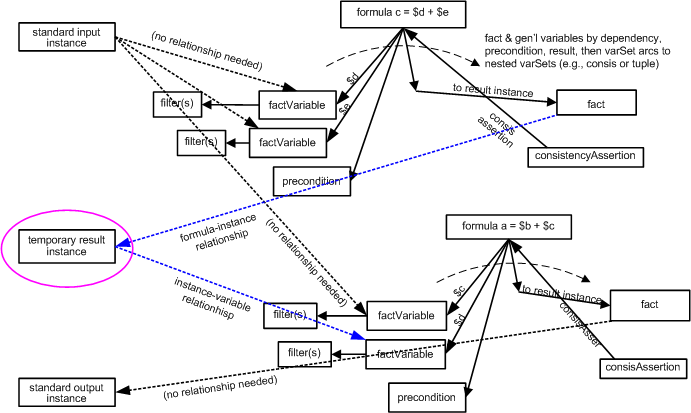Example 16: Chaining C = D + E to A = B + C by instance relationships

<formula:decimals>
0
</formula:decimals>
<formula:aspects>
<formula:concept>
<formula:qname>
test:c
</formula:qname>
</formula:concept>
</formula:aspects>
</formula:formula>
(1) This formula produces c = d + e, where c is produced into temp-c-instance.
The declaration of temp-c-instance, the scratch-pad for holding the output of formula (1), to become the input to formula (2)'s variable_c.
Arc s connecting formula (1) above to temp-c-instance, and formula (2)'s variable_c to bind its input to the fact(s) in temp-c-instance, effecting the "chaining". The fact, temp:c, gets produced in temp-c-instance, by (1), and consumed from temp-c-instance by variable_c of (2).
<formula:decimals>
0
</formula:decimals>
<formula:aspects>
<formula:concept>
<formula:qname>
test:a
</formula:qname>
</formula:concept>
</formula:aspects>
</formula:formula>
(2) This formula produces a = b + c, using variable c from the temporary instance produced by (1).
The fact variables for above formulas. Note that no general variables are used in this example.
(The concept name filters are omitted from this example figure, but are available in the SVN testcase file abcde-formula1.xml in directory 60400 Chaining-Processing.)
The output fact A is produced into the standard XBRL instance, and may normally be saved in an output XBRL instance file. However the output fact C is in the temp-c-instance, and would not be saved in the standard output file. If it is desirable to save C in the standard output instance, it would have to be copied there, because instance chaining must completely produce the facts of one instance before they can be consumed by other variables in another, preventing use of the standard output instance as both a fact production destination and a source for consuming facts to produce more results into itself.

# 19 Tuple output production overview

Tuples are produced in an output instance by use of the ``` <tuple:tuple> ```, which is similar to the ``` <formula:formula> ``` except that it does not specify rules for value, accuracy, and any of the fact item aspects. Nested tuple and item elements may be produced in the tuple by ``` <tuple:tuple> ``` and ``` <formula:formula> ``` elements that have a variables-scope relationship from the parent ``` <tuple:tuple> ```, which conveys the variables of the parent variable-set and a variable name for the parent tuple. The variable name from the parent tuple conveys the location aspect via the variables-scope relationship, so that the child tuple or item may use that in a ``` <tuple:location> ``` rule ``` @source``` attribute, in the manner of Figure 36.

Figure 36: Formulae to produce output XBRL instance tuples.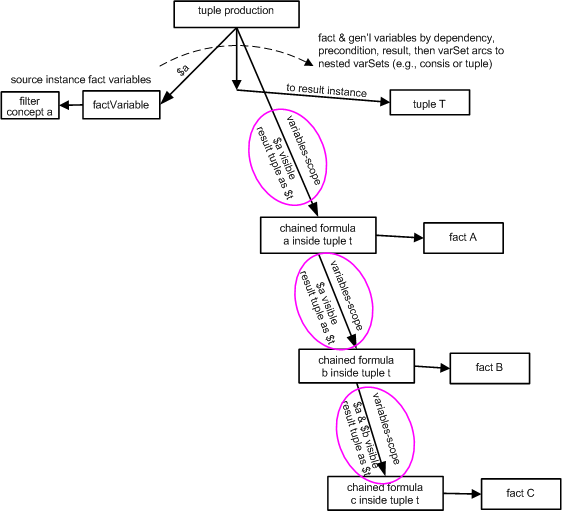This example shows a simple test case to generate a tuple from testcase input facts. The usual tuple use case tends to be fairly elobarate and involved, such as to process input Global Ledger instances and output normalized, summed, or verified Global Ledger instances. A simpler test case is provided in SVN directory 60500 FormulaTuples-Processing, regionProductSalesToTuple-formula.xml, where a dimensional example input instance is converted to a non-dimensional tuple output instance. Here is a much simpler test case, taub-formula1.xml of the same testcases directory, that contrives to demonstrate tuple output from a simple single-item input.

• There are facts in the input instance providing a single value for each input period, such as:
<test:a contextRef="c1" unitRef="u1" decimals="0">
1001
</test:a>
• The output instance produces a double-nesting of tuples with derived contrived fact items as follows:
<test:t>
<test:a contextRef="c1" unitRef="u1" decimals="0">
1001
</test:a>
<test:u>
<test:b contextRef="c1" unitRef="u1" decimals="0">
1002
</test:b>
</test:u>
</test:t>

Figure 37: Example producing nested tuples each with a fact item.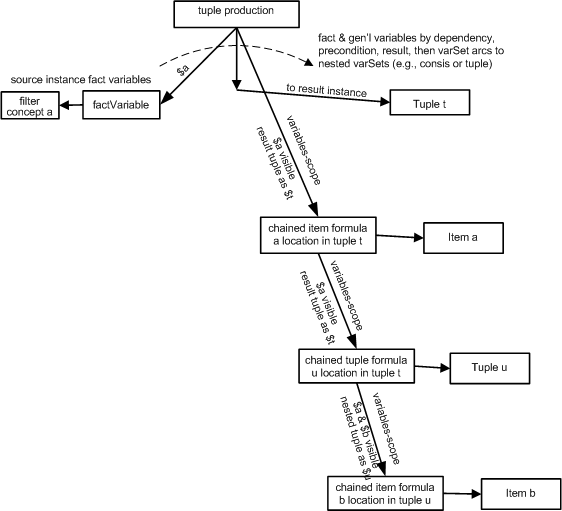Example 17: Summing aspect-matched facts across three input instances

<formula:aspects>
<formula:concept>
<formula:qname>
test:t
</formula:qname>
</formula:concept>
<!-- as a tuple, no other aspects are applicable -->
</formula:aspects>
</tuple:tuple>
(1) tuple t formula evaluates when test:a is input to variable inputInstanceFactItemA; there are two of these test:a's, so there will be two tuple t's resulting (in the test case taub-formula1.xml).
Tuple t (1) of the output instance, and variable test:a from the input instance are both visible to nested item A formula (2)
<formula:decimals>
0
</formula:decimals>
<formula:aspects>
<formula:concept>
<formula:qname>
test:a
</formula:qname>
</formula:concept>
<tuple:location source="outputInstanceTupleT"/>
</formula:aspects>
</formula:formula>
(2) formula formulaItemA produces a single fact item ``` <test:a> ``` located inside tuple T.
formulaItemA's tuple output result, and fact item A in tuple t output result, and variable from the input instance, all are visible to formulaTupleU (3)
<formula:aspects>
<formula:concept>
<formula:qname>
test:u
</formula:qname>
</formula:concept>
<tuple:location source="outputInstanceTupleT"/>
<!-- as a tuple, no other aspects are applicable -->
</formula:aspects>
</tuple:tuple>
Formula (3) formulaTupleU produces nested tuple U located inside tuple T in sequence after item a.
The result of (3) formulaTupleU is made visible, by variables-scope relationship, to formulaItemB (4) below, so that it can locate fact item B inside tuple U. By this relationship, tuple U and all bound variables for (3) formulaTupleU are made visible to formula (4).
<formula:decimals>
0
</formula:decimals>
<formula:aspects>
<formula:concept>
<formula:qname>
test:b
</formula:qname>
</formula:concept>
<tuple:location source="outputInstanceTupleU"/>
</formula:aspects>
</formula:formula>
(4) Formula to produce a single test:b fact item = \$itemA + 1, located inside tuple u.
There is only a single fact variable, which binds to the input facts ``` <test:a> ```. Each evaluation of this fact variable produces the tuple t, nested item a, nested tuple u, and u's nested item b.

## 19.1 Chaining with variable scope relationships

This example shows the same case of two formulas related by chaining, A = B + C, and C = D + E, as used in Section 18.1 for instances chaining, but here implemented using variables-scope chaining.

• Formula 1 (A=B+C): Result is A, factVariables B & C factVariable B is from input XBRL instance factVariable C is variables-scope related to the result of formula (2), C
• Formula 2 (C=D+E): Result is C, factVariables D & E, result is variables-scope related to (1), the formula for A

Figure 38: Chaining by variable scope relationships.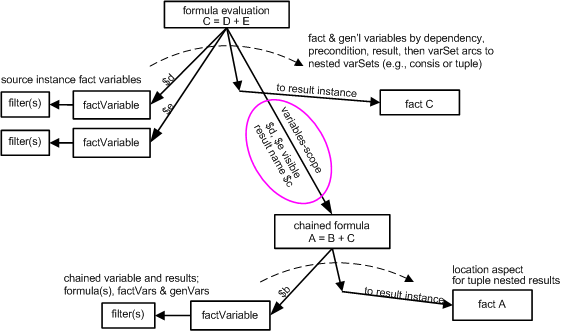Example 18: Chaining C = D + E to A = B + C by variables-scope relationships

<formula:decimals>
0
</formula:decimals>
<formula:aspects>
<formula:concept>
<formula:qname>
test:c
</formula:qname>
</formula:concept>
</formula:aspects>
</formula:formula>
(1) formula produces a single test:c result fact item
formulaC's result (1) is made visible to formulaA (2), below
<formula:decimals>
0
</formula:decimals>
<formula:aspects>
<formula:concept>
<formula:qname>
test:a
</formula:qname>
</formula:concept>
</formula:aspects>
</formula:formula>
(2) formula produces a = b + c, where the term c is bound by variables-scope relationship to the output of formula (1) above
These are the factVariables that are bound to fact items from the input instance.
(The concept name filters are omitted from this example figure, but are available in the SVN testcase file abcde-formula1.xml in directory 60600 VariablesScope-Processing.)
The output fact A is produced into the standard XBRL instance, and may normally be saved in an output XBRL instance file. However the output fact C is in the temp-c-instance, and would not be saved in the standard output file. If it is desirable to save C in the standard output instance, it would have to be copied there, because instance chaining must completely produce the facts of one instance before they can be consumed by other variables in another, preventing use of the standard output instance as both a fact production destination and a source for consuming facts to produce more results into itself.

# Appendix A Intellectual property status (non-normative)

This document and translations of it may be copied and furnished to others, and derivative works that comment on or otherwise explain it or assist in its implementation may be prepared, copied, published and distributed, in whole or in part, without restriction of any kind, provided that the above copyright notice and this paragraph are included on all such copies and derivative works. However, this document itself may not be modified in any way, such as by removing the copyright notice or references to XBRL International or XBRL organizations, except as required to translate it into languages other than English. Members of XBRL International agree to grant certain licenses under the XBRL International Intellectual Property Policy (www.xbrl.org/legal).

This document and the information contained herein is provided on an "AS IS" basis and XBRL INTERNATIONAL DISCLAIMS ALL WARRANTIES, EXPRESS OR IMPLIED, INCLUDING BUT NOT LIMITED TO ANY WARRANTY THAT THE USE OF THE INFORMATION HEREIN WILL NOT INFRINGE ANY RIGHTS OR ANY IMPLIED WARRANTIES OF MERCHANTABILITY OR FITNESS FOR A PARTICULAR PURPOSE.

The attention of users of this document is directed to the possibility that compliance with or adoption of XBRL International specifications may require use of an invention covered by patent rights. XBRL International shall not be responsible for identifying patents for which a license may be required by any XBRL International specification, or for conducting legal inquiries into the legal validity or scope of those patents that are brought to its attention. XBRL International specifications are prospective and advisory only. Prospective users are responsible for protecting themselves against liability for infringement of patents. XBRL International takes no position regarding the validity or scope of any intellectual property or other rights that might be claimed to pertain to the implementation or use of the technology described in this document or the extent to which any license under such rights might or might not be available; neither does it represent that it has made any effort to identify any such rights. Members of XBRL International agree to grant certain licenses under the XBRL International Intellectual Property Policy (www.xbrl.org/legal).

# Appendix B Acknowledgements (non-normative)

This document could not have been written without the contributions of many people including the participants in the Formula Working Group.

# Appendix C Document history (non-normative)

DateAuthorDetails
12 October 2011Herm Fischer

Initial draft

06 December 2011Herm Fischer

Added sections from Section 13 to Section 19.1.

16 December 2011Herm Fischer

Added UML class diagrams Section 6, updated Section 3 and Section 16.

# Appendix D Errata corrections in this document

This appendix contains a list of the errata corrections that have been incorporated into this document. This represents all those errata corrections that have been approved by the XBRL International Formula Working Group up to and including 21 December 2011.

No errata have been incorporated into this document.